• ## G2O

2018-12-21 15:03:32
前言 ... 本节我们将深入介绍视觉slam中的主流优化方法——图优化（graph-based ...关于g2o，我13年写过一个文档，然而随着自己理解的加深，越发感觉不满意。本着对读者更负责任的精神，本文给大家重新讲一...
前言
转载自高博士的文章:
https://www.cnblogs.com/gaoxiang12/p/5244828.html
本节我们将深入介绍视觉slam中的主流优化方法——图优化（graph-based optimization）。下一节中，介绍一下非常流行的图优化库：g2o。
关于g2o，我13年写过一个文档，然而随着自己理解的加深，越发感觉不满意。本着对读者更负责任的精神，本文给大家重新讲一遍图优化和g2o。除了这篇文档，读者还可以找到一篇关于图优化的博客： http://blog.csdn.net/heyijia0327 那篇文章有作者介绍的一个简单案例，而本文则更注重对图优化和g2o的理解与评注。
本节主要介绍图优化的数学理论，下节再讲g2o的组成方式及使用方法。
预备知识：优化
图优化本质上是一个优化问题，所以我们先来看优化问题是什么。
优化问题有三个最重要的因素：目标函数、优化变量、优化约束。一个简单的优化问题可以描述如下：
minF(x)
其中x为优化变量，而F(x)为优化函数。此问题称为无约束优化问题，因为我们没有给出任何约束形式。由于slam中优化问题多为无约束优化，所以我们着重介绍无约束的形式。
当F(x)有一些特殊性质时，对应的优化问题也可以用一些特殊的解法。例如，F(x)为一个线性函数时，则为线性优化问题（不过线性优化问题通常在有约束情形下讨论）。反之则为非线性优化。对于无约束的非线性优化，如果我们知道它梯度的解析形式，就能直接求那些梯度为零的点，来解决这个优化：
                           dF/dx=0

梯度为零的地方可能是函数的极大值、极小值或者鞍点。由于现在F(x)的形式不确定，我们只好遍历所有的极值点，找到最小的作为最优解。但是我们为什么不这样用呢？因为很多时候F(x)
的形式太复杂，导致我们没法写出导数的解析形式，或者难以求解导数为零的方程。因此，多数时候我们使用迭代方式求解。从一个初值x0出发，不断地导致当前值附近的，能使目标函数下降的方式（反向梯度），然后沿着梯度方向走出一步，从而使得函数值下降一点。这样反复迭代，理论上对于任何函数，都能找到一个极小值点。
迭代的策略主要体现在如何选择下降方向，以及如何选择步长两个方面。主要有 Gauss-Newton （GN）法和 Levenberg-Marquardt （LM）法两种，它们的细节可以在维基上找到，我们不细说。请理解它们主要在迭代策略上有所不同，但是寻找梯度并迭代则是一样的。
图优化
所谓的图优化，就是把一个常规的优化问题，以图（Graph）的形式来表述。
图是什么呢？
图是由顶点（Vertex）和边（Edge）组成的结构，而图论则是研究图的理论。我们记一个图为G={V,E}
，其中V为顶点集，E为边集。
顶点没什么可说的，想象成普通的点即可。
边是什么呢？一条边连接着若干个顶点，表示顶点之间的一种关系。边可以是有向的或是无向的，对应的图称为有向图或无向图。边也可以连接一个顶点（Unary Edge，一元边）、两个顶点（Binary Edge，二元边）或多个顶点（Hyper Edge，多元边）。最常见的边连接两个顶点。当一个图中存在连接两个以上顶点的边时，称这个图为超图（Hyper Graph）。而SLAM问题就可以表示成一个超图（在不引起歧义的情况下，后文直接以图指代超图）。
怎么把SLAM问题表示成图呢？
SLAM的核心是根据已有的观测数据，计算机器人的运动轨迹和地图。假设在时刻k
，机器人在位置xk处，用传感器进行了一次观测，得到了数据zk
。传感器的观测方程为：
zk=h(xk)
由于误差的存在，zk不可能精确地等于h(xk)
，于是就有了误差：ek=zk−h(xk)
那么，如果我们以xk
为优化变量，以minxFk(xk)=‖ek‖为目标函数，就可以求得xk
的估计值，进而得到我们想要的东西了。这实际上就是用优化来求解SLAM的思路。
你说的优化变量xk，观测方程zk=h(xk)等等，它们具体是什么东西呢？
这个取决于我们的参数化（parameterazation）。x
可以是一个机器人的Pose（6自由度下为 4×4的变换矩阵T 或者 3自由度下的位置与转角[x,y,θ]，也可以是一个空间点（三维空间的[x,y,z]或二维空间的[x,y]）。相应的，观测方程也有很多形式，如：
机器人两个Pose之间的变换；
机器人在某个Pose处用激光测量到了某个空间点，得到了它离自己的距离与角度；
机器人在某个Pose处用相机观测到了某个空间点，得到了它的像素坐标；
同样，它们的具体形式很多样化，这允许我们在讨论slam问题时，不局限于某种特定的传感器或姿态表达方式。
我明白优化是什么意思了，但是它们怎么表达成图呢？
在图中，以顶点表示优化变量，以边表示观测方程。由于边可以连接一个或多个顶点，所以我们把它的形式写成更广义的 zk=h(xk1,xk2,…)
，以表示不限制顶点数量的意思。对于刚才提到的三种观测方程，顶点和边是什么形式呢？
机器人两个Pose之间的变换；——一条Binary Edge（二元边），顶点为两个pose，边的方程为T1=ΔT⋅T2。
机器人在某个Pose处用激光测量到了某个空间点，得到了它离自己的距离与角度；——Binary Edge，顶点为一个2D Pose：[x,y,θ]T
和一个Point：[λx,λy]T，观测数据是距离r和角度b，那么观测方程为：
[rb]=⎡⎣⎢⎢⎢(λx−x)2+(λy−y)2‾‾‾‾‾‾‾‾‾‾‾‾‾‾‾‾‾‾‾√tan−1(λy−yλx−x)−θ⎤⎦⎥⎥⎥
机器人在某个Pose处用相机观测到了某个空间点，得到了它的像素坐标；——Binary Edge，顶点为一个3D Pose：T和一个空间点x=[x,y,z]T，观测数据为像素坐标z=[u,v]T。那么观测方程为：
z=C(Rx+t)
C为相机内参，R,t为旋转和平移。
举这些例子，是为了让读者更好地理解顶点和边是什么东西。由于机器人可能使用各种传感器，故我们不限制顶点和边的参数化之后的样子。比如我（丧心病狂地在小萝卜身上）既加了激光，也用相机，还用了IMU，轮式编码器，超声波等各种传感器来做slam。为了求解整个问题，我的图中就会有各种各样的顶点和边。但是不管如何，都是可以用图来优化的。
图优化怎么做
现在让我们来仔细看一看图优化是怎么做的。假设一个带有n
条边的图，其目标函数可以写成：
min∑k=∑ek(xk,zk)TΩkek(xk,zk)(7)
关于这个目标函数，我们有几句话要讲。这些话都是很重要的，请读者仔细去理解。
e函数在原理上表示一个误差，是一个矢量，作为优化变量xk和zk符合程度的一个度量。它越大表示xk越不符合zk。但是，由于目标函数必须是标量，所以必须用它的平方形式来表达目标函数。最简单的形式是直接做成平方：e(x,z)Te(x,z)。进一步，为了表示我们对误差各分量重视程度的不一样，还使用一个信息矩阵 Ω来表示各分量的不一致性。

信息矩阵 Ω
是协方差矩阵的逆，是一个对称矩阵。它的每个元素Ωi,j作为eiej的系数，可以看成我们对ei,ej这个误差项相关性的一个预计。最简单的是把Ω
设成对角矩阵，对角阵元素的大小表明我们对此项误差的重视程度。
这里的xk可以指一个顶点、两个顶点或多个顶点，取决于边的实际类型。所以，更严谨的方式是把它写成ek(zk,xk1,xk2,…)，但是那样写法实在是太繁琐，我们就简单地写成现在的样子。由于zk是已知的，为了数学上的简洁，我们再把它写成ek(xk)的形式。
于是总体优化问题变为n
条边加和的形式：
minF(x)=∑k=1nek(xk)TΩkek(xk)
重复一遍，边的具体形式有很多种，可以是一元边、二元边或多元边，它们的数学表达形式取决于传感器或你想要描述的东西。例如视觉SLAM中，在一个相机Pose Tk
处对空间点xk进行了一次观测，得到zk，那么这条二元边的数学形式即为
ek(xk,Tk,zk)=(zk−C(Rxk+t))TΩk(zk−C(Rxk−t))
单个边其实并不复杂。
现在，我们有了一个很多个节点和边的图，构成了一个庞大的优化问题。我们并不想展开它的数学形式，只关心它的优化解。那么，为了求解优化，需要知道两样东西：一个初始点和一个迭代方向。为了数学上的方便，先考虑第k
条边ek(xk)吧。
我们假设它的初始点为x˜k
，并且给它一个Δx的增量，那么边的估计值就变为Fk(x˜k+Δx)，而误差值则从 ek(x˜) 变为 ek(x˜k+Δx)。首先对误差项进行一阶展开：
ek(x˜k+Δx)≈ek(x˜k)+dekdxkΔx=ek+JkΔx
这是的Jk是ek关于xk的导数，矩阵形式下为雅可比阵。我们在估计点附近作了一次线性假设，认为函数值是能够用一阶导数来逼近的，当然这在Δx很大时候就不成立了。
于是，对于第k条边的目标函数项，有：
进一步展开：
Fk(x˜k+Δx)=≈==ek(x˜k+Δx)TΩkek(x˜k+Δx)(ek+JkΔx)TΩk(ek+JΔx)eTkΩkek+2eTkΩkJkΔx+ΔxTJTkΩkJkΔxCk+2bkΔx+ΔxTHkΔx
在熟练的同学看来，这个推导就像(a+b)2=a2+2ab+b2
一样简单（事实上就是好吧）。最后一个式子是个定义整理式，我们把和Δx无关的整理成常数项 Ck ，把一次项系数写成 2bk ，二次项则为 Hk
（注意到二次项系数其实是Hessian矩阵）。
请注意 Ck
实际就是该边变化前的取值。所以在xk发生增量后，目标函数Fk项改变的值即为
ΔFk=2bkΔx+ΔxTHkΔx.
我们的目标是找到Δx
，使得这个增量变为极小值。所以直接令它对于Δx
的导数为零，有：
dFkdΔx=2b+2HkΔx=0⇒HkΔx=−bk
所以归根结底，我们求解一个线性方程组：
HkΔx=−bk
如果把所有边放到一起考虑进去，那就可以去掉下标，直接说我们要求解
HΔx=−b.
原来算了半天它只是个线性的！线性的谁不会解啊！
读者当然会有这种感觉，因为线性规划是规划中最为简单的，连小学生都会解这么简单的问题，为何21世纪前SLAM不这样做呢？——这是因为在每一步迭代中，我们都要求解一个雅可比和一个海塞。而一个图中经常有成千上万条边，几十万个待估计参数，这在以前被认为是无法实时求解的。
那为何后来又可以实时求解了呢？
SLAM研究者逐渐认识到，SLAM构建的图，并非是全连通图，它往往是很稀疏的。例如一个地图里大部分路标点，只会在很少的时刻被机器人看见，从而建立起一些边。大多数时候它们是看不见的（就像后宫怨女一样）。体现在数学公式中，虽然总体目标函数F(x)
有很多项，但某个顶点xk就只会出现在和它有关的边里面！
这会导致什么？这导致许多和xk无关的边，比如说ej，对应的雅可比Jj就直接是个零矩阵！而总体的雅可比J中，和xk有关的那一列大部分为零，只有少数的地方，也就是和xk顶点相连的边，出现了非零值。相应的二阶导矩阵H中，大部分也是零元素。这种稀疏性能很好地帮助我们快速求解上面的线性方程。出于篇幅我们先不细说这是如何做到的了。稀疏代数库包括SBA、PCG、CSparse、Cholmod等等。g2o正是使用它们来求解图优化问题的。
要补充一点的是，在数值计算中，我们可以给出雅可比和海塞的解析形式进行计算，也可以让计算机去数值计算这两个阵，而我们只需要给出误差的定义方式即可。
流形等一下老师！上面推导还有一个问题！
很好，小萝卜同学，请说说是什么问题。
我们在讨论给目标函数F(x)一个增量Δx时，直接就写成了F(x+Δx)。但是老师，这个加法可能没有定义！
小萝卜同学看到了一个严重的问题，这确实是在先前的讨论中忽略掉了。由于我们不限制顶点的类型，x在参数化之后，很可能是没有加法定义的。
最简单的就是常见的四维变换矩阵T或者三维旋转矩阵R。它们对加法并不封闭，因为两个变换阵之和并不是变换阵，两个正交阵之和也不是正交阵。它们乘法的性质非常好，但是确实没有加法，所以也不能像上面讨论的那样去求导。
但是，如果图优化不能处理SE(3)或SO(3)中的元素，那将是十分令人沮丧的，因为SLAM要估计的机器人轨迹必须用它们来描述啊。回想我们先前讲过的李代数知识。虽然李群 SE(3)
和 SO(3) 是没有加法的，但是它们对应的李代数 ??(3),??(3)
有啊！ 数学一点地说，我们可以求它们在正切空间里的流形上的梯度！如果读者觉得理解困难，我们就说，通过指数变换和对数变换，先把变换矩阵和旋转矩阵转换成李代数，在李代数上进行加法，然后再转换到原本的李群中。这样我们就完成了求导。
这样的好处是我们完全不用重新推导公式。这件事比我们想的更加简单。在程序里，我们只需重新定义一个优化变量x
的增量加法即可。如果x是一个SE(3)里的变换矩阵，我们就遵守刚才讲的李代数转换方式。当然，如果x是其他什么奇怪的东东，只要定义了它的加法，程序就会自动去计算如何求它的雅可比。
核函数
又是核函数！——学过机器学习课程的同学肯定要这样讲。
但是很遗憾，图优化中也有一种核函数。 引入核函数的原因，是因为SLAM中可能给出错误的边。SLAM中的数据关联让科学家头疼了很长时间。出于变化、噪声等原因，机器人并不能确定它看到的某个路标，就一定是数据库中的某个路标。万一认错了呢？我把一条原本不应该加到图中的边给加进去了，会怎么样？
嗯，那优化算法可就慒逼了……它会看到一条误差很大的边，然后试图调整这条边所连接的节点的估计值，使它们顺应这条边的无理要求。由于这个边的误差真的很大，往往会抹平了其他正确边的影响，使优化算法专注于调整一个错误的值。
于是就有了核函数的存在。核函数保证每条边的误差不会大的没边，掩盖掉其他的边。具体的方式是，把原先误差的二范数度量，替换成一个增长没有那么快的函数，同时保证自己的光滑性质（不然没法求导啊！）。因为它们使得整个优化结果更为鲁棒，所以又叫它们为robust kernel（鲁棒核函数）。
很多鲁棒核函数都是分段函数，在输入较大时给出线性的增长速率，例如cauchy核，huber核等等。当然具体的我们也不展开细说了。
核函数在许多优化环境中都有应用，博主个人印象较深的时当年有一大堆人在机器学习算法里加各种各样的核，我们现在用的svm也会带个核函数。
小结
最后总结一下做图优化的流程。
选择你想要的图里的节点与边的类型，确定它们的参数化形式；
往图里加入实际的节点和边；
选择初值，开始迭代；
每一步迭代中，计算对应于当前估计值的雅可比矩阵和海塞矩阵；
求解稀疏线性方程HkΔx=−bk    ，得到梯度方向；
继续用GN或LM进行迭代。如果迭代结束，返回优化值。
实际上，g2o能帮你做好第3-6步，你要做的只是前两步而已。下节我们就来尝试这件事。



展开全文• 文章目录g2o学习记录（2）官方文档的阅读及理解前言g2o描述和介绍基本定义SLAM而言的例子超图g2o的目的（超）图可嵌入优化问题超图优化问题最小二乘优化关于线性化方程组结构的思考流形上的最小二乘法稳健最小二乘库...
文章目录g2o学习记录（2）官方文档的阅读及理解前言g2o描述和介绍基本定义SLAM而言的例子超图g2o的目的（超）图可嵌入优化问题超图优化问题最小二乘优化关于线性化方程组结构的思考流形上的最小二乘法稳健最小二乘库的概览g2o类图优化问题的表示线性化问题的构造与表示初始化计算误差线性化方程组构建线性方程组更新Levenberg-Marquardt求解器(Solvers)扩展知识：舒尔补Actionsg2o工具g2o命令行界面g2o Viewerg2o增量(incremental)g2o_incremental使用示例Plug-in Architecture(插件架构)2D SLAM:一个例子确定状态变量约束的建模增量参数化的选择误差函数的设计整理(Putting things together)总结
g2o学习记录（2）官方文档的阅读及理解
前言
本文是自己的理解，并不能保证准确性，如果有误，请大家指出。参考文献在后给出，最主要是根据新版本博文(1)中介绍安装的里面的doc进行阅读和使用，期间会穿插旧版本，比如高翔14讲中的g2o旧版本（无unit_test)的g2o的代码测试。本文仅做自己的学习记录，欢迎大家交流讨论。系列文以g2o下的doc为主，其他书籍是辅助作用。
由于CSDN采用Latex渲染器KATEX，行内公式编号的tag命令不能过多指定，为了保证编号的准确性，我便舍弃了\begin{align}和\end{align}进行公式的优化，也舍弃了我原本在mardown语法中编写公式使用\begin{eqnarray}和\end{eqnarray}嵌套组，请大家阅读的时候知道=号其实是对齐的就成。
g2o描述和介绍
基本定义
g2o是一个C++框架，用于执行非线性最小二乘问题的优化，它是一个图优化工具。 非线性最小二乘问题又恰恰是由许多误差项和组成，如果把最小二乘以图的形式表示，那么顶点是优化变量，而边是误差项。
SLAM而言的例子
对于SLAM中使用而言，这里截取两本书的例子，希望有个大致的了解。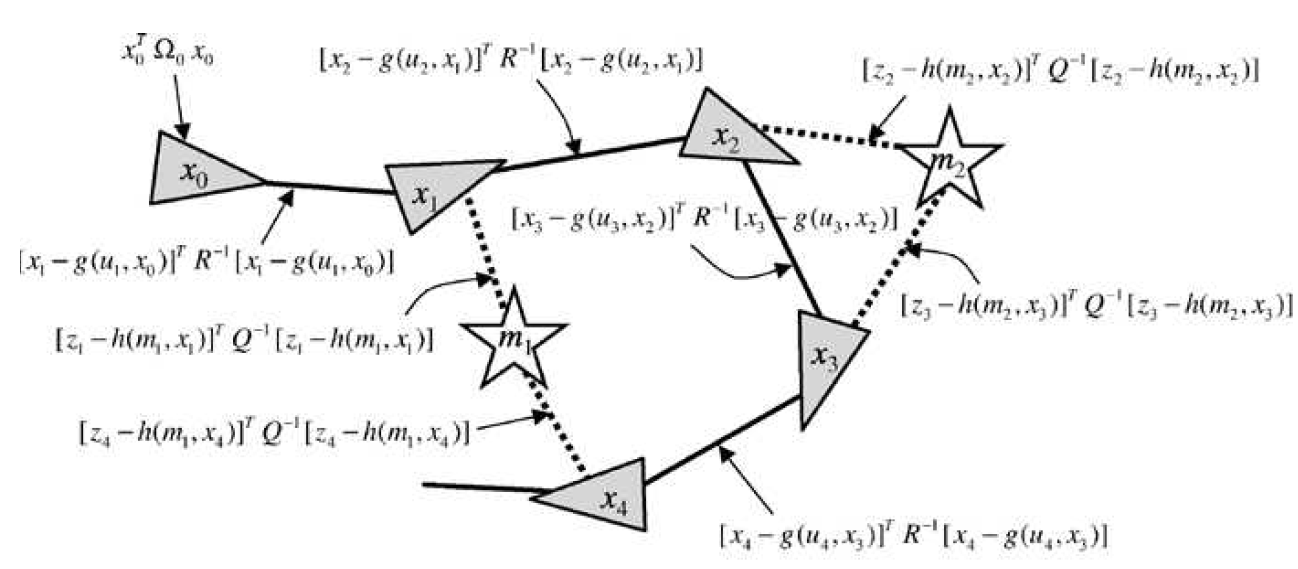图1。GraphSLAM算法示例。摘自Probabilistic Robotics书的338页码。个人不太建议中文版，翻译得水准有失偏颇，术语有些存在不准确性。当然有条件和时间，可以中英文对照阅读。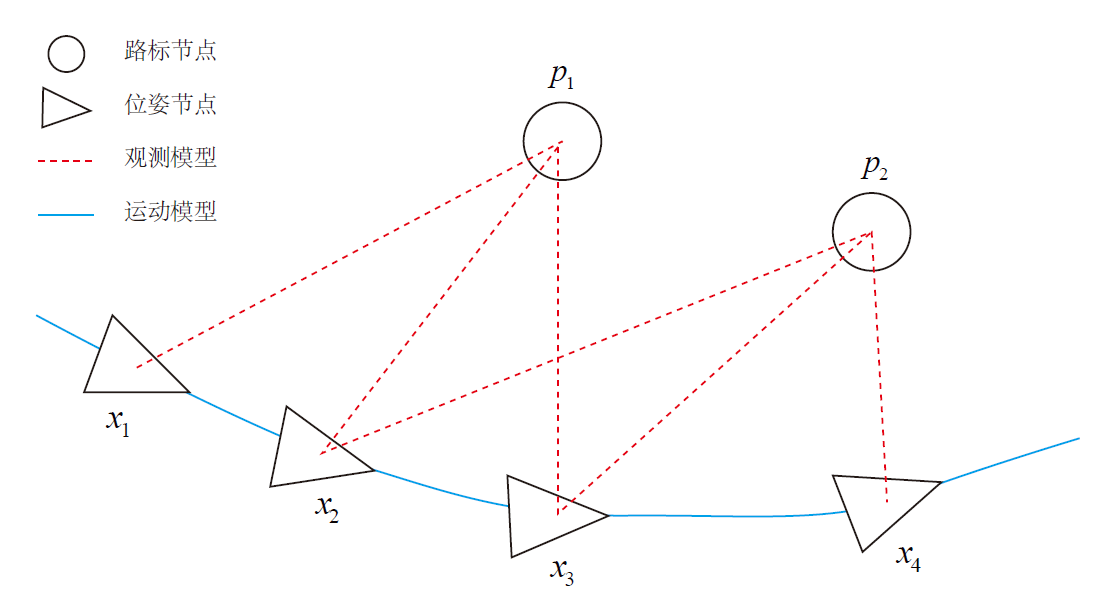图2。图优化例子。摘自高翔SLAM十四讲第六章第122页。三角形表示位姿，圆形是图像观察到的路标点。

我本身不太希望，我写的就像是介绍graph slam，主要是理论部分对我现在来说，只需要了解大概，因为我是使用它而不是深入改造它。
我们就说图1，对于图1来看，有四个机器人位置和两个地图特征。图中节点，是机器人位姿和特征的位置。比如$x_0,x_1,x_2,x_3,x_4$，以及$m_1,m_2$。实线就是机器人的位姿，虚线是表示在该位姿下看到的特征。对于graph slam来说，它会认为，每一个连接都是二次方非线性约束。这些约束会被表示为测量和运动模型的负对数似然，也被称为信息约束。对于graph slam来说，这些约束加入图后并不重要。
它最小化的就是下面的这些约束，这构成了一个最小二乘问题。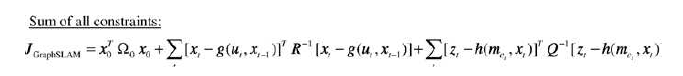因为它要找到在这个约束下，产生最大似然地图和对应的一系列机器人位姿。为什么是最大似然？这种问题我不会过于展开，这在SLAM十四讲会讲到（你可以类比该书的第108页的公式$(6.12)$，求导过程我目前不会展示），因为我们要在已知的测量条件下，求出最大的可能性，概率机器人这本书还是以EKF讲解，所以这里面还涉及了贝叶斯公式，这里先不细说，而且我自己也还没看完，以后有时间再讨论。
现在我们要知道的是，上面的最小二乘的问题被构建成了图优化问题，你主要是记住，要优化的是节点，误差项是边。节点是我们要估计的，误差项是我们定义的。比如我们估计一系列的数据，是否符号一元二次方程$f(x)=ax^2+bx+c$，我们要估计的是$a,b,c$则作为图的节点，而误差项，就是测量值和估量值之间的差值。相当于$y-f(x)$，我们要迭代求解得到这个测量值和估量值之间的差值最小的时候，也就是求到了满足这么多点的一元二次方程的$a,b,c$，这就是一个图求解的问题。
而在SLAM中，我们要求解的是位姿，然后误差项是重投影误差，或者光度误差或者其他自定义的误差，最主要的思想是利用g2o.ceres-solver等工具，使得求出一组最佳的位姿，使得这些误差最小。
好的，这些就让我们过了这个话题。我们只需要知道g2o是一个求解工具，它将我们定义的那种误差的最小二乘问题能求解出来，求得符合的最佳位姿就够了。我们主要还是在g2o的了解和使用上，现在我不会去过多考虑g2o由其扩展的Graph SLAM的理论部分。
超图
在g2o文档中定义了一个超图（Hyper Graph），超图（Hyper Graph）是图的扩展，其中边可以连接多个节点而不仅仅是两个节点，甚至只连接一个都可以，比如下图。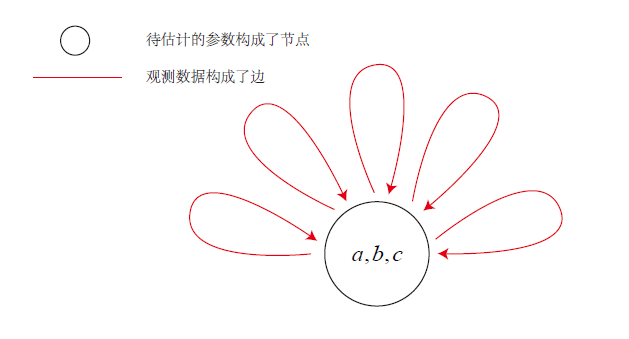SLAM十四讲，123页，曲线拟合对应的图优化模型。

对于超边(Hyper Edge)和超图（Hyper Graph）的定义我也不是很喜欢，大体知道它就是一个图，只不过不是一般的图那样，边只能连接两个节点。
在机器人和计算机视觉中的若干问题需要找到关于一组参数的误差函数的最佳优化。 例子包括SLAM和BA等流行应用。
g2o的目的

为了提供易于扩展且易于使用的图形优化通用库，可以轻松应用于不同的问题，
为想要了解SLAM或BA的人提供易于阅读的实现，该实现侧重于问题规范的相关细节。
尽可能地提供最先进的性能。

（超）图可嵌入优化问题
Graph-Embeddable在理解起来可能大家和我第一次看的时候差不多想法，这个是什么东西？估计很多人知道Graph Embedding（图嵌入），比如这篇论文Exploring the Semantic Content of Unsupervised Graph Embeddings: An Empirical Study。
这篇论文把Graph Embedding定义为：图嵌入是一系列机器学习模型，它们学习图中顶点的潜在表示，目的是将矢量空间中没有固有表示的复杂图转换为其元素的低维矢量表示。
这个意思估计也不太好懂，我觉得这篇博文Graph Embedding 综述描述倒是比较清晰些，定义为：将网络的节点和向量之间建立一个一一对应的关系，并且保留网络的一些拓扑性质。
这里有一篇关于Graph Embedding的论文综述，也描述了这部分的知识。在机器学习领域最近倒是很喜欢把降维以embedding来指代。
我觉得这里已经扯远了，作者的意思很可能表达的可嵌入性超图，意味着，我们可以把一个多维度的优化问题转换成图问题，这减少了问题的求解难度（其实感觉意思表达也不错，我们把问题降低了维度一样，使得问题求解变得容易了）。
超图优化问题
我们知道最小二乘最小化问题可以通过以下等式描述：
$\rm F( x)= \sum_{k \in\mathcal{C}}\underbrace{e_k(x_k, z_{k})^T \Omega_{k}e_k( x_k, z_{k})}_{F_{k}}\tag{1}$
$x^*=\mathop{\arg\min}_{\rm x} \rm F(x)\tag{2}$
其中：

${\rm x} = ({\rm x}_1^T,\cdots,{\rm x}_n^T)^T$是参数向量，其中每一个$\rm x_i$表示通用参数块。
${\rm x}_k = ({\rm x}_{k_1}^T,\cdots,{\rm x}_{k_q}^T)^T \sub ({\rm x}_1^T,\cdots,{\rm x}_n^T)^T$是第$k$个约束涉及的参数子集
${\rm z}_k$和$\Omega_k$分布表示与${\rm x}_k$中参数相关的约束的均值和信息矩阵
$e_k(x_k, z_{k}）$是一个向量误差函数，用于测量${\rm x}_k$中参数块满足约束${\rm z}_k$的程度。 当${\rm x}_k$和${\rm x}_j$与约束完全匹配时为0。 作为示例，如果具有测量函数$\hat{\rm z}_k={\rm h}_k({\rm x}_k)$，其在给定${\rm x}_k$中的节点的实际配置的情况下生成合成测量$\hat{\rm z}_k$。 然后直接的(straightforward)误差函数是${\rm e(x}_k,{\rm z}_k)={\rm h}_k({\rm x}_k)-{\rm z}_k$。

为简单起见，定义如下：
${\rm e}_k({\rm x}_k, {\rm z}_{k}) \overset{\mathrm{def.}}= \; {\rm e}_k({\rm x}_k) \; \overset{\mathrm{def.}}= \; {\rm e}_k(\rm x)\tag{3}$
请注意，每个参数块和每个误差函数都可以张成(span)不同的空间。 这种形式的问题可以通过有向超图来有效地表示。 图的节点$i$表示参数块${\rm x}_i\in {\rm x}_k$，并且节点${\rm x}_i\in {\rm x}_k$中的超边表示涉及${\rm x}_k$中的所有节点的约束。 在超边具有大小为2的情况下（个人理解是边只连接了两个图的节点），超图变为普通图。 下图显示了超图和目标函数之间的映射示例。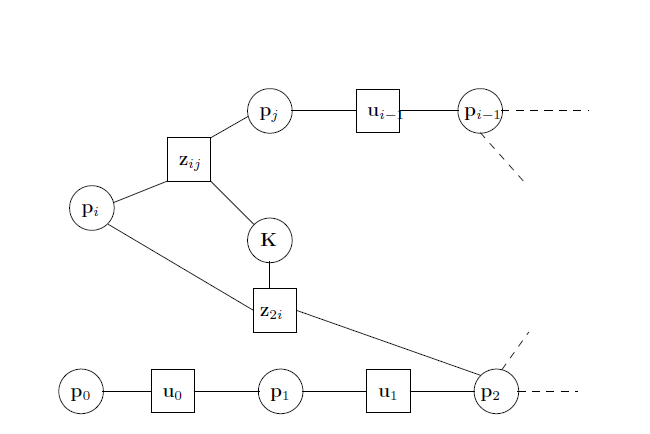摘自g2o的doc文档，说明如何通过超图表示目标函数。

上图说明一个小SLAM问题的一部分。 在这个例子中，我们假设测量函数由一些未知的校准参数$K$控制。机器人位姿由变量${\rm p}_{1:n}$表示。 这些变量通过方框所描绘的约束${\rm z}_{ij}$连接。 例如，通过匹配在激光参考系（the laser reference frame）中的附近激光扫描来产生约束。 然而，激光匹配和机器人位姿之间的关系取决于传感器在机器人上的位置，其由校准参数$K$建模。相反，随后的机器人位姿通过由测距法测量（odometry measurements，实际上也是我们说的视觉里程计）引起的二元约束来连接。 这些测量是在移动机器人为基的坐标系（移动坐标系）所产生的（如果是vSLAM，你可以看成是相机拍摄到的物体的以相机坐标系得到的路标测量值）。
最小二乘优化
如果已知参数的良好初始猜测 $\breve{\rm x}$，则公式2的数值解可以通过使用流行的Gauss-Newton（高斯牛顿）或Levenberg-Marquardt（莱文贝格-马夸特方法）算法获得。

作者推荐阅读numerical recipes的第二版15.5部分了解LM算法，不过目前已经出现第三版，而中文版C++版本也有源码可以下。

这个想法【指的GN和LM】是通过围绕当前初始猜测 $\breve{\rm x}$的一阶泰勒展开来近似误差函数：
$e_{k}(\breve{\rm x}_k + \Delta x_k) = e_{k}(\breve{x} + \Delta x)\tag{4}$
$\simeq e_{k} + J_{k} \Delta x \tag{5}$
其中${\rm J}_k$是在$\breve{\rm x}$ 和${\rm e}_k\overset{\mathrm{def.}}={\rm e}_k(\breve{\rm x})$中计算的${\rm e}_k$的雅可比行列式。 用等式（1）中的${\rm F}_k$ 的误差项【这里描述应该是$e_k(x_k, z_{k}）$，前面有等式（3）说明了标记的同等性，这是下面7到8替换获得】由等式（5）代替。 我们获得
${{\rm F}_{k}(\breve{\rm x} + \Delta{\rm x}) }\tag{6}$
$= {\rm e}_{k}(\breve{\rm x} + \Delta{\rm x})^T \Omega_{k} {\rm e}_{k}(\breve{\rm x} + \Delta x) \tag{7}$
$\simeq \left({\rm e}_{k} + {\rm J}_{k} \Delta{\rm x} \right)^T \Omega_{k} \left({\rm e}_{k} + {\rm J}_{k} \Delta{\rm x} \right) \tag{8}$
$= \underbrace{{\rm e}_{k}^T \Omega_{k}{\rm e}_{k}}_{\mathrm{c}_{k}} + 2\underbrace{{\rm e}_{k}^T \Omega_{k} {\rm J}_{k}}_{{\rm b}_{k}} \Delta{\rm x} +\Delta{\rm x} ^T \underbrace{{\rm J}_{k}^T \Omega_{k}{\rm J}_{k}}_{{\rm H}_{k}} \Delta{\rm x} \tag {9}$
$=\mathrm{c}_{k} + 2 {\rm b}_{k} \Delta{\rm x} + \Delta{\rm x} ^T {\rm H}_{k} \Delta{\rm x} \tag{10}$
通过这种局部近似，可以重写式（1）中给出的函数${\rm F}(x)$如下所示：
${\rm F}(\breve{\rm x} + \Delta {\rm x}) = \sum_{k \in\mathcal{C}} {\rm F}_{k}(\breve{\rm x}+ \Delta {\rm x}) \tag{11}$
$\simeq \sum_{k \in\mathcal{C}} \mathrm{c}_{k} + 2 {\rm b}_{k} \Delta{\rm x} + \Delta{\rm x} ^T {\rm H}_{k} \Delta{\rm x}\tag{12}$
$=\mathrm{c} + 2 {\rm b}^T \Delta{\rm x} + \Delta{\rm x} ^T {\rm H} \Delta{\rm x} \tag{13}$
通过设置$c=\sum c_k$,${\rm b}=\sum{\rm b}_k$和${\rm H}=\sum{\rm H}_k$，就可以从等式（12）中获得等式（13）的二次形式。 它可以通过求解线性方程组在$\Delta{\rm x}$处被最小化
${\rm H}\Delta {\rm x}^*=-{\rm b}\tag{14}$
矩阵$\rm H$是方程组的信息矩阵，并且通过构造是稀疏的，仅在通过约束连接的块之间具有非零。 其非零块的数量是约束数加上节点数的两倍。 这允许解决方程式(14)采用稀疏Cholesky分解或预处理共轭梯度（PCG）等有效方法。 稀疏Cholesky分解的高效实现可以在开源的软件包中找到，如CSparse或CHOLMOD。 然后通过将初始猜测添加到计算的增量来获得线性化解
${\rm x}^*=\breve{\rm x}+\Delta {\rm x}^*\tag{15}$
流行的Gauss-Newton算法迭代了等式（13）中的线性化， 等式（14）中的解和等式15中的更新步骤。 在每次迭代中，先前的解决方案用作线性化点和初始猜测。
Levenberg-Marquardt（LM）算法是Gauss-Newton的非线性变体，它引入阻尼因子(damping factor)和备用动作（backup actions）来控制收敛。
我个人不太喜欢这篇doc中描述的backup actions，显得不是很清晰。文中也就出现过一次。我们只需要知道LM是高斯牛顿的变体，不过是加入了阻尼系数，采用了一定的策略，在运行过程中更改，以便稳定收敛，后期我打算观看高斯牛顿和LM算法方面的书籍，进行自我总结学习，大体思路了解一下差不多就可以了。
LM不是直接解决方程式14，而是解决了它的阻尼版本：
$({\rm H}+\lambda \rm I)\Delta {\rm x}^*=-{\rm b}\tag{16}$
其中$\lambda$是一个阻尼因子：$\lambda$越大$\Delta \rm x$越小。 这对于在非线性表面的情况下控制步长是有用的。 LM算法背后的想法是动态控制阻尼因子。 在每次迭代时，都会监视新配置的error。 如果新error低于前一个error，则下一次迭代将减少$\lambda$。 否则，恢复解决方案并增加$\lambda$。【关于LM的描述，建议可以查阅相关博客进行了解，主要是判断实际减少和近似模型减少的比值，在SLAM十四讲第113页也有描述，相对描述得浅显易懂些】
g2o中实现的LM算法，其参考SBA: A Software Package for Generic Sparse Bundle Adjustment。
上述过程是多变量函数最小化的一般方法。 然而，一般方法假设参数$\rm x$的空间是欧几里德空间，这对于SLAM或BA等几个问题无效。 这可能导致次优解决方案。
关于线性化方程组结构的思考
按照方程式(13)中，通过对一组矩阵和向量求和来获得矩阵$\rm H$和向量$\rm b$，每个约束对应一个矩阵和向量。 如果我们设置${\rm b}_k={\rm J}_k^T\Omega_k{\rm e}_k$和${\rm H}_k={\rm J}_k^T\Omega_k{\rm J}_k$，我们可以将$\rm H$和$\rm b$重写为
${\rm b}=\sum_{k \in\mathcal{C}} {\rm b}_{ij}\tag{17}$
${\rm H}=\sum_{k \in\mathcal{C}} {\rm H}_{ij}\tag{18}$
每个约束都将通过加数项为方程组做出贡献。 此加数的结构取决于误差函数的雅可比行列式。 由于约束的误差函数仅取决于节点${\rm x}_i\in {\rm x}_k$的值，因此方程（5）中的雅可比行列式具有以下形式【这一部分的想要彻底理解，可以阅读SLAM十四讲第248页，关于稀疏性和边缘化问题，图文化显示得很不错】：
${\rm J}_{k}= \left({\bold 0} \cdots {\bold 0} \; {\rm J}_{k_1} \; \cdots \; {\rm J}_{k_i} \;\cdots {\bold 0} \; \cdots\; {\rm J}_{k_q} {\bold 0} \cdots {\bold 0} \right)\tag{19}$
其中${\rm J}_{k_i}=\frac{\partial {\rm e}({\rm x}_k)}{\partial {\rm x}_{k_i}}$是关于由第$k$个超边连接的节点的误差函数的导数，相对于参数块${\rm x}_{k_i}\in {\rm x}_k$。
通过等式（9），我们得到块矩阵${\rm H}_{ij}$的结构如下：
${\rm H}_k=\begin{pmatrix}\ddots\\&{\rm J}_{k_1}^T\Omega_k{\rm J}_{k_1}&\cdots&{\rm J}_{k_1}^T\Omega_k{\rm J}_{k_i}&\cdots&{\rm J}_{k_1}^T\Omega_k{\rm J}_{k_q}&\\&{\rm J}_{k_i}^T\Omega_k{\rm J}_{k_1}&\cdots&{\rm J}_{k_i}^T\Omega_k{\rm J}_{k_i}&\cdots&{\rm J}_{k_i}^T\Omega_k{\rm J}_{k_q}&\\&{\rm J}_{k_q}^T\Omega_k{\rm J}_{k_1}&\cdots&{\rm J}_{k_q}^T\Omega_k{\rm J}_{k_i}&\cdots&{\rm J}_{k_q}^T\Omega_k{\rm J}_{k_q}&\\&&&&&&\ddots\end{pmatrix}\tag{20}$
${\rm b}_k=\begin{pmatrix}\vdots \\{\rm J}_{k_1}\Omega_k{\rm e}_k\\\vdots \\{\rm J}_{k_i}\Omega_k{\rm e}_k\\\vdots \\{\rm J}_{k_q}\Omega_k{\rm e}_k\\\vdots\end{pmatrix}\tag{21}$
为简单起见，我们省略了零块。 可能会注意到矩阵$\mathrm H$的块结构是超图的邻接矩阵。 另外，Hessian $\mathrm H$是对称矩阵，因为所有${\rm H}_k$都是对称的。 连接$q$个顶点的单个超边将在Hessian中引入$q^2$非零块，超边对应于连接的节点每对$<{\rm x}_{k_i},{\rm x}_{k_j}>$。
流形上的最小二乘法
为了处理张成非欧几里德空间的参数块，通常在流形上应用误差最小化【实际上我想认为这说的是李群，这是一种流形】。 流形是一个数学空间，在全局尺度（global scale）内不一定是欧几里德，但可以在局部尺度（local scale）上看作欧几里德（Introduction to Smooth Manifolds，该书的相关页还有此处链接）。
例如，在SLAM问题的上下文(context)中，每个参数块${\rm x}_i$由平移向量${\rm t}_i$和旋转分量$\alpha_i$组成。 平移向量${\rm t}_i$明显形成欧几里德空间。 与此相反，旋转分量$\alpha_i$张成非欧几里德2D或3D旋转组$SO(2)$或$SO(3)$。

SO(2)是二维旋转，SO（3）是三维旋转

为了避免奇点，通常以过度参数化的方式描述这些空间，例如通过旋转矩阵或四元数。 这些过参数化的表示直接应用等式15破坏过参数化引起的约束。 过参数化导致额外的自由度，从而在解决方案中引入误差。 为了克服这个问题，可以使用旋转的极小表示（如3D中的欧拉角）。 然而，这将受到奇点的影响【万向锁请了解一下】。
另一种想法是将underlying space（底空间）视为一个流形，并定义一个算子$\boxplus$将欧几里得空间中的局部变量(local variation)$\Delta {\rm x}$映射到流形上的变量,$\Delta {\rm x}\mapsto {\rm x}\; \boxplus \Delta{\rm x}$。

在Bourbaki, Nicolas的Elements of mathematics: Theory of sets 著作中定义"topological space" is an underlying structure of the “Euclidean space” structure，意思是拓扑空间是欧氏空间的 underlying structure。我可以认为底空间是拓扑空间的意思。这里面作者以underlying space描述，委实让我理解起来的时候却是有点难度，我只能理解为在欧氏空间中的拓扑空间的意思。

此类流行空间优化的具体细节阅读A framework for sparse, non-linear least squares problems on manifolds的1.3部分【此文我还没得阅读】。 使用此运算符，可以将新的误差函数定义为
$\breve{\rm e}_k(\Delta \tilde{\rm x}_k) \overset{\mathrm{def.}}={\rm e}_k(\tilde{\rm x}_k\boxplus\Delta\tilde{\rm x}_k)\tag{22}$
$={\rm e}_k(\tilde{\rm x}\boxplus\Delta\tilde{\rm x})\simeq \breve{\rm e}_k+\tilde{\rm J}_k\Delta{\tilde{\rm x}}\tag{23}$
其中 $\breve{\rm x}$ 张成原始的过度参数化空间，例如四元数。 $\Delta \tilde{\rm x}$项是初始位置周围$\breve{\rm x}$的小增量，并以最小表示来表示。 $SO(3)$的常见选择是使用单位四元数的向量部分。 更详细地说，可以将增量$\Delta \tilde{\rm x}$表示为6D向量 $\Delta \tilde{\rm x}^T=(\Delta\tilde{\rm t}\;\tilde{\rm q}^T)$，其中$\Delta\tilde{\rm t}$表示平移，而$\Delta\tilde{\rm q}^T$是单位四元数的向量部分表示3D旋转。 相反，$\breve{\rm x}=(\breve{\rm t}^T\;\breve{q}^T)$使用四元数$\breve{q}^T$来对旋转部分编码。 因此，算子$\boxplus$可以被表示为首先通过将$\Delta\tilde{\rm q}$转换为完全四元数$\Delta {\rm q}$然后将变换$\Delta{\rm x}^T=(\Delta{\rm t}\;\Delta{\rm q}^T)$应用于$\breve{\rm x}$【从22到23式】。

估计在上下标处会迷糊，我是这样认为，$\breve{\rm x}$这种表示是初始猜测的表示。$\tilde{\rm x}$的上标这种表示微小增量。后面也有需要这种表示的说明需要很好的了解才行。

在描述误差最小化的等式中，这些操作可以很好地由$\boxplus$算子封装。 雅可比矩阵$\tilde{\rm J}_k$可以表达为:
$\tilde {\rm J}_{k} = \left. \frac{\partial {\rm e}_{k}(\breve{\rm x} \boxplus \Delta \tilde{\rm x})} {\partial \Delta \tilde{\rm x}} \right|_{\Delta \tilde{\rm x}={\bold 0}}\tag{24}$
由于在前面的等式中，$\breve{\rm e}$仅依赖于$\Delta \tilde{\rm x}_{k_i}\in \Delta \tilde{\rm x}_k$，我们可以进一步扩展如下：
$\tilde {\rm J}_{k} = \left. \frac{\partial {\rm e}_{k}(\breve{\rm x} \boxplus \Delta \tilde{\rm x})} {\partial \Delta \tilde{\rm x}} \right|_{\Delta \tilde{\rm x}=\bold 0}\tag{25}$
$= \left(\bold 0 \cdots \bold 0 \; \tilde {\rm J}_{k_1} \; \cdots \; \tilde {\rm J}_{k_i} \;\cdots \bold 0\; \cdots\; \tilde {\rm J}_{k_q} \bold 0 \cdots \bold 0 \right).\tag{26}$
通过简单的表示法扩展，我们设
$\tilde {\rm J}_{k_i} = \left. \frac{\partial {\rm e}_{k}(\breve{\rm x} \boxplus \Delta \tilde{\rm x})} {\partial \Delta \tilde{\rm x}_{k_i}} \right|_{\Delta \tilde{\rm x}={\bold 0}}\tag{27}$
通过简单的表示法扩展，我们可以在等式（8）和等式（11）中插入等式（23）。 这导致以下增量：
$\tilde{\rm H}\Delta\tilde{\rm x}^*=-\tilde{\rm b}\tag{28}$
由于增量$\Delta\tilde{\rm x}^*$是在初始猜测$\breve {\rm x}$的局部欧几里得环境中计算的，因此需要通过$\boxplus$操作符将它们重新映射到原始冗余空间中。 因此，等式（15）的更新规则变成了
${\rm x}^*=\breve{\rm x} \boxplus \Delta\tilde{\rm x}^*\tag{29}$
总之，在流形上形式化最小化问题包括首先由公式(28）计算围绕初始猜测的局部欧几里得近似的一组增量，然后通过公式（29）累加全局非欧几里得空间中的增量。
注意，在流形表示上计算的线性方程组具有与在欧几里德空间上计算的线性方程组相同的结构。可以很容易地从非流形版本推导出图最小化的流形版本，只需要定义一个⊞算子及其雅克比矩阵 $\tilde{\rm J}_{k_i}$ 相应的参数块。g2o提供了用于在流型空间上数值计算雅可比的工具。这要求用户仅实现误差函数和⊞操作符。
g2o没有解决非流型情况，因为该问题已经包含在流型中。但是，为了获得最大的性能和准确性，建议the user to implement analytic Jacobians, once the system is functioning with the numeric ones。
这句建议不知道怎么翻译才准确表达，个人理解这句话，作者的意思是，雅克比的矩阵，在已知一部分数值的时候，可以通过数学公式推导得到计算的雅克比的最终代数式子（可能仅需要几个数值就好），而不是用g2o帮你计算，可以加速性能和准确性。
稳健最小二乘
可选地，最小二乘优化可以是稳健的。 请注意，方程式（1）中的误差项具有以下形式：
${\rm F}_k={\rm e}_k^T\Omega_k{\rm e}_k=\rho_2(\sqrt{{\rm e}_k^T\Omega_k{\rm e}_k})\tag{30}$
其中$\rho_2(x):=x^2$
因此，误差向量${\rm e}_k$对$\rm F$具有二次影响，因此单个潜在异常值将具有主要的负面影响。 为了使更加离群的鲁棒性，二次误差函数$\rho_2$可以用更稳健的成本函数代替，该成本函数对较小的误差进行较小的权重。 在g2o中，可以使用Huber成本函数$\rho_H$。
$\rho_H:=\begin{cases}x^2&\text{if}\; |x|<b\\2b|x|-b^2&\text{else},\end{cases}\tag{31}$
对于小$|x|$，它是二次的,但对于大$|x|$而言是线性的。 与其他更强大的成本函数相比，Huber内核的优势在于它仍然是凸的，因此不会在$\bold F$ 中引入新的局部最小值。
下面是摘自多视图几何第二版的616页的一些成本函数的成本函数，概率密度函数，衰减系数。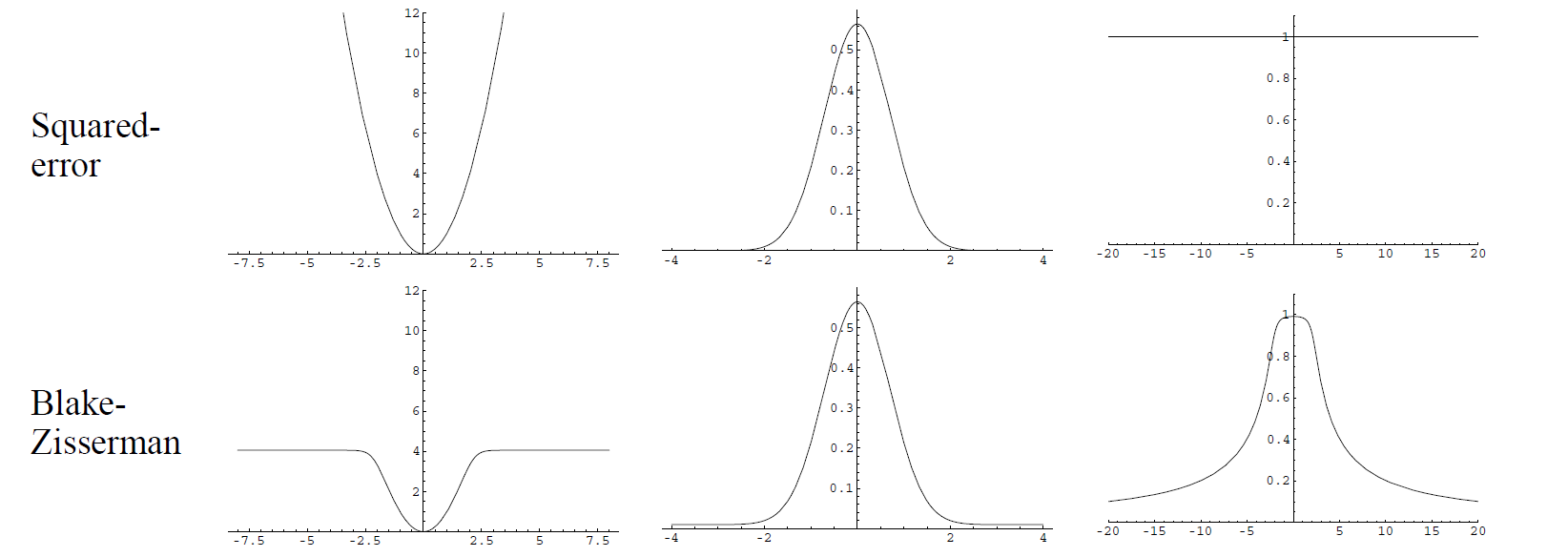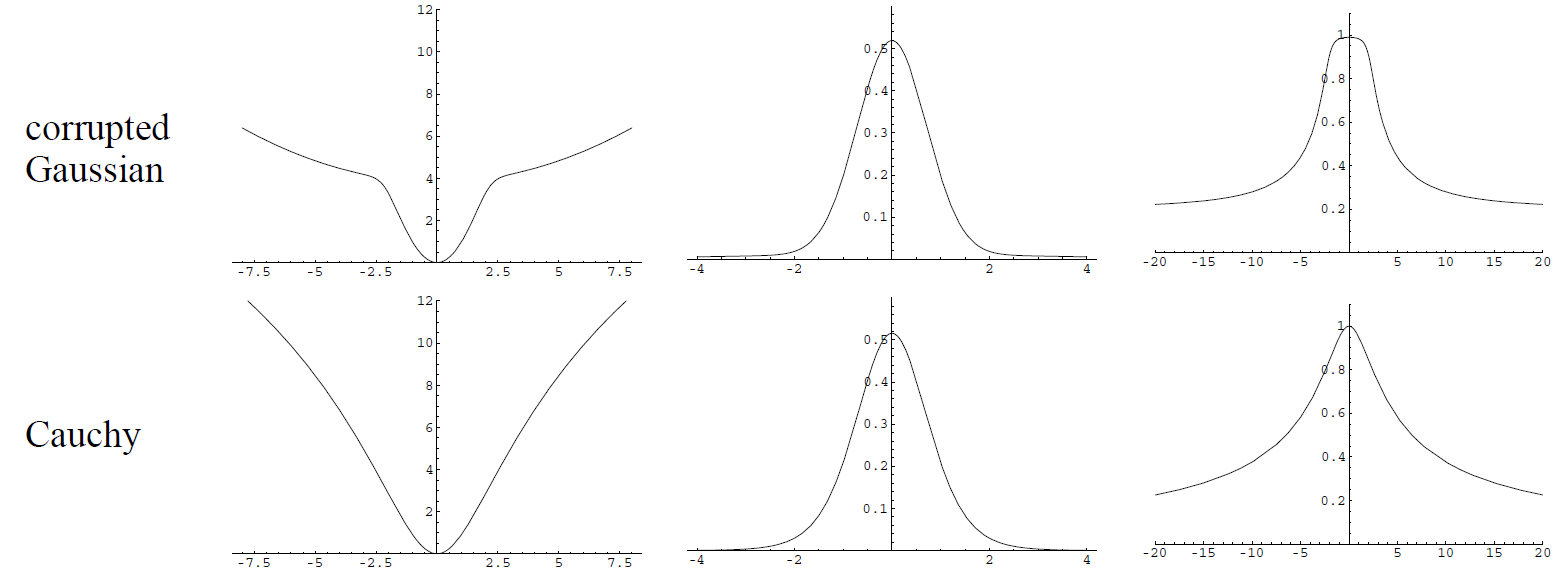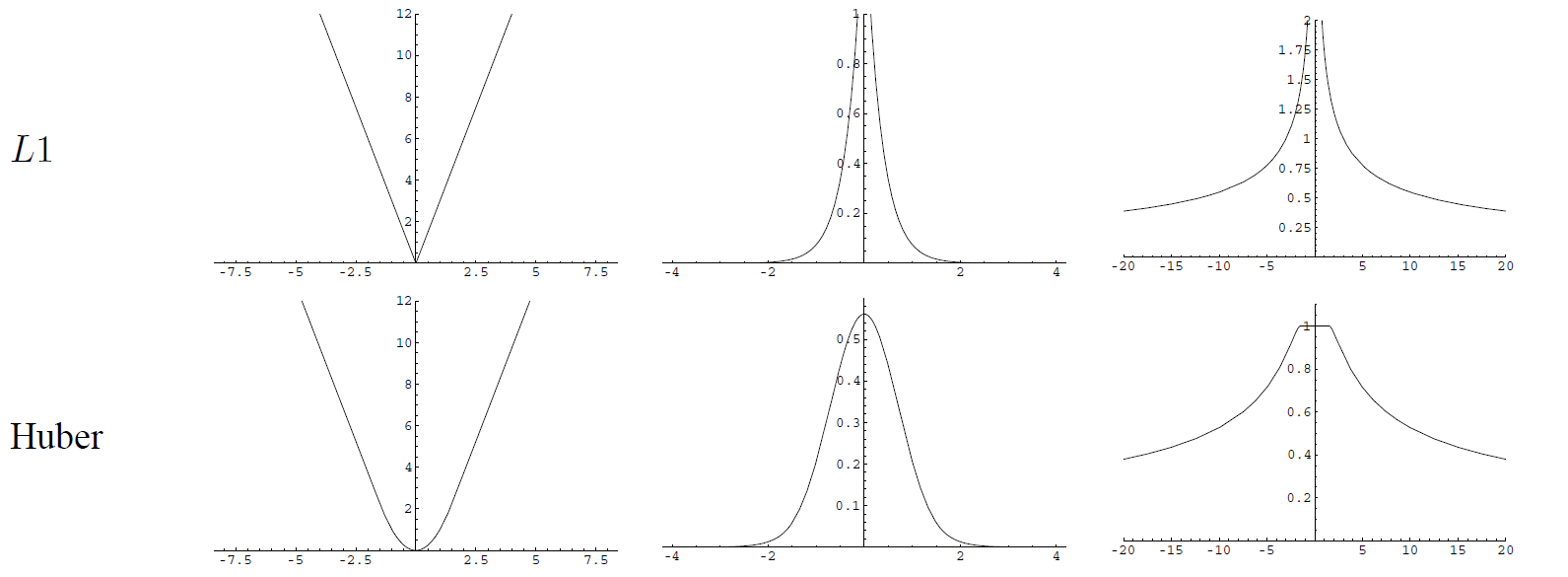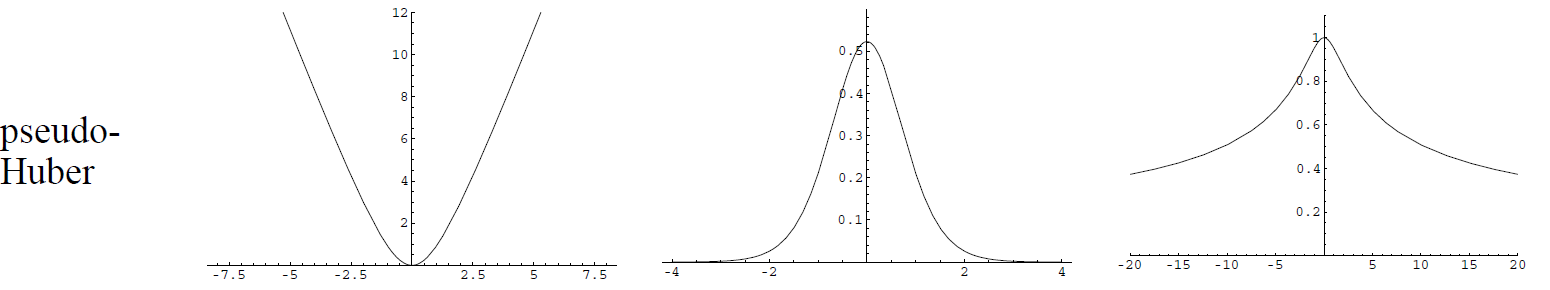这些函数以后有时间再仔细说明。回到正题，在实践中，我们不需要修改等式（1）。 相反，应用以下方案。 首先，像往常一样计算误差${\rm e}_k$。 然后，${\rm e}_k$被加权版本$w_k{\rm e}_k$取代:
$(w_k{\rm e}_k)^T\Omega_k(w_k{\rm e}_k)=\rho_H\left(\sqrt{{\rm e}_k^T\Omega_k{\rm e}_k}\right)\tag{32}$
其中，权重$w_k$如下计算
$w_k=\frac{\sqrt{\rho_H(||{\rm e}_k||_\Omega)}}{||{\rm e}_k||_\Omega}\tag{33}$
其中$||{\rm e}_k||_\Omega:=\sqrt{{\rm e}_k^T\Omega_k{\rm e}_k}$。
在g2o中，用户具有细粒度控制，可以单独启用/禁用每个边的稳健成本函数（参见下文）。
库的概览
从上面的部分可以清楚地看出，图优化问题完全由以下定义：

图中顶点的类型（即参数块$\{{\rm x}_i\}$。对于每个顶点的类型必须指定：

内部参数化(internal parameterization)的定义域(domain) $\text{Dom}({\rm x}_i)$，
增量$\Delta {\rm x}_i$的定义域 $\text{Dom}(\Delta{\rm x}_i)$,
$\boxplus:\text{Dom}({\rm x}_i)\times \text{Dom}(\Delta{\rm x}_i)\rightarrow \text{Dom}({\rm x}_i)$，应用于增量$\Delta{\rm x}_i$到先前的方案${\rm x}_i$.

每种类型的超边${\rm e}_k$的误差函数：$\text{Dom}(\Delta{\rm x}_{k_1})\times\text{Dom}(\Delta{\rm x}_{k_2})\times \cdots \text{Dom}(\Delta{\rm x}_{k_q})\rightarrow\text{Dom}({\rm z}_k)$当扰动估计${\rm x}_k\boxplus \Delta{\rm x}_k$完全满足约束${\rm z}_k$时应该为零。

默认情况下，Jacobians由g2o的框架以数字方式计算。 然而，为了在特定实现中实现最大性能，可以指定误差函数和流形运算符的雅可比行列式。
g2o类图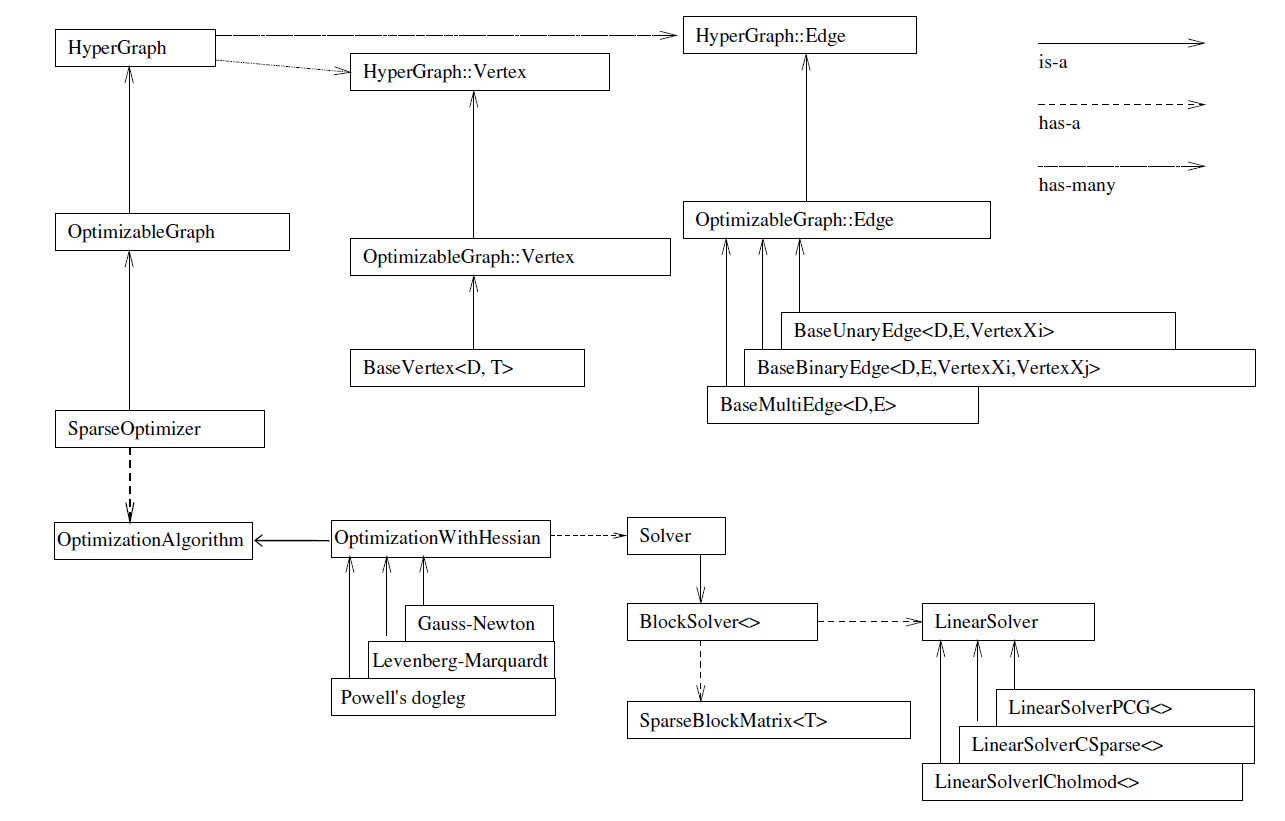优化问题的表示
g2o中利用通用的超图结构来表示问题实例（在hyper_graph.h中定义）。 此通用超图表专门用于表示OptimizableGraph类的优化问题，该类在optimizable_graph.h中定义。 在OptimizableGraph中，内部类OptimizableGraph :: Vertex和OptimizableGraph :: Edge用于表示通用超边和超顶点。 虽然可以通过直接扩展这些类来完成特定的实现，但g2o提供了一个模板特化，它自动实现了方程组(system)必须使用的大多数方法。
这些类是BaseVertex和BaseUnaryEdge，BaseBinaryEdge和BaseMultiEdge。

BaseVertex模拟参数块${\rm x}_i$和相应流形$\Delta{\rm x}_i$的维数，因此它可以使用其编译时（意味着效率）已知布局的存储块（blocks of memory）。 此外，它实现了一些映射运算符来存储Hessian和线性化问题的参数块，以及一堆可用于保存/恢复图部分的先前值。 将由$\rm v$表示的扰动$\Delta {\rm x}_i$应用于成员变量_estimate的方法oplusImpl（double * v）应该被实现。 这是$\boxplus$运算符。 此外，必须指定应将顶点的内部状态设置为$\bold 0$的setToOriginImpl()。
template <int D, typename T>
class BaseVertex : public OptimizableGraph::Vertex


BaseUnaryEdge是一个模拟一元超边(unary hyper-edge)的模板类，可用于表示先验。 它通过linearizeOplus方法的实现免费提供雅可比行列式的计算。 它需要指定（单个）顶点${\rm x}_i$的类型，以及误差${\rm e}({\rm x}_k)$的类型和维度作为模板参数。 将误差${\rm e}({\rm x}_k)$的结果存储在成员Eigen :: Matrix _error中的函数computeError应该被实现。
  template <int D, typename E, typename VertexXi>
class BaseUnaryEdge : public BaseEdge<D,E>


BaseBinaryEdge是一个对二元约束的建模的模板类，即${\rm e}_k({\rm x}_{k_1},{\rm x}_{k_2})$形式的误差函数。 它提供与BaseUnaryEdge相同的功能，并且需要指定以下模板参数：节点${\rm x}_{k_1}$和${\rm x}_{k_2}$的类型以及测量的类型和维度。 同样，它通过linearizeOplus方法的默认实现实现了数值形式的雅可比行列式。 同样，computeError应该在派生类中实现。

  template <int D, typename E, typename VertexXi, typename VertexXj>
class BaseBinaryEdge : public BaseEdge<D, E>


BaseMultiEdge是一个模板类，它以${\rm e}_k({\rm x}_{k_1},{\rm x}_{k_2},\cdots,{\rm x}_{k_q})$的形式模拟多顶点约束。 它提供与上述类型相同的功能，并且只需要将测量的类型和维度指定为模板参数。特化类（specialized class，应指已经制定了模板是何种类型的类）应该注意将连接的顶点的大小调整为正确的大小$q$。 这个类依赖于动态内存，因为太多参数是未知的，如果您需要针对特定问题的高效实现，可以自己编程。 数值化的雅可比矩阵是免费的（意思是g2o提供了默认的运算代码），但您应该像往常一样在派生类中实现computeError

  template <int D, typename E>
class BaseMultiEdge : public BaseEdge<D,E>

简而言之，定义新问题实例所需要做的就是从上面列出的那些类中派生一组类，每类参数块便是一个类，每种类型的（超）边便是一个类（意思是你需要定义节点和边，分别用类来表示）。 总是试着从最适合你的类派生出来。 如果你想看一个简单的例子，请看vertex_se2和edge_se2。 这两种类型定义了一个简单的2D图SLAM问题，就像许多SLAM论文中描述的那样。
当然，对于构造的每种类型，您还应该定义read和write函数，以便将数据读取和写入流。 最后，一旦定义了新类型，要启用加载和保存新类型，您应该将其“注册”到工厂。 这可以通过registerType函数将字符串标记分配给新类型来轻松完成。 这应该在加载所有文件之前调用一次。
为此，g2o提供了一个简单的宏来执行类到工厂的注册。 有关示例，请参见清单1，完整示例可在types_slam2d.cpp中找到。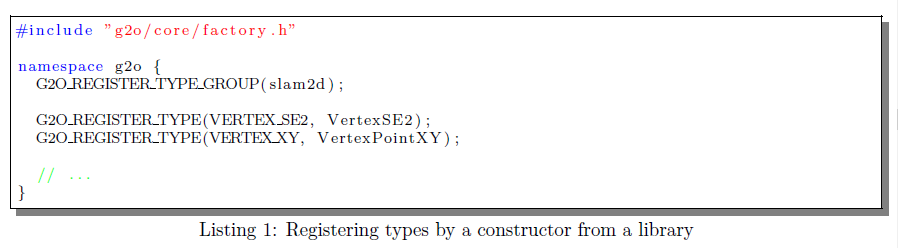给宏G2O_REGISTER_TYPE的第一个参数指定了某个顶点/边已知的标签。 g2o将在加载文件时使用此信息并将当前图保存到文件中。 在清单1给出的示例中，分别使用VertexSE2和EdgeSE2类注册标记VERTEX_SE2和EDGE_SE2。
此外，宏G2O_REGISTER_TYPE_GROUP允许声明类型组。 如果我们使用工厂来构造类型，那么这是必要的，我们必须强制我们的代码链接到特定的类型组。 否则链接器可能会删除我们的库，因为我们没有显式使用包含我们类型的库提供的任何符号。 声明使用特定类型库并因此强制执行链接是由G2O_USE_TYPE_GROUP命名的宏完成的。
线性化问题的构造与表示
可以将构造和解决方案分成单独的步骤，这些步骤是迭代的。

初始化优化（仅在第一次迭代之前）。
计算每个约束的误差向量。
线性化每个约束。
构建线性方程组。
更新Levenberg-Marquardt阻尼系数。

在以下部分中，我们将介绍这些步骤。

个人认为，这个步骤的流程将LM说得倒是蛮清楚了。

初始化
SparseOptimizer类提供了几种初始化底层(underlying)数据结构的方法。

前面我有指出，underlying一般是对应于拓扑空间，所以这个意思我理解是初始化几种拓扑空间的基（关于基我不想太多描述，学过线性代数的人应该会基础）。

initializeOptimization()方法采用顶点子集或边子集，这些边将被考虑用于下一次优化运行。 此外，可以考虑所有顶点和边进行优化。 我们分别引用当前被视为活动顶点(active vertices)和边的顶点和边。
在初始化过程中，优化器（optimizer）为每个活动顶点分配一个临时索引(temporary index)。 该临时索引对应于Hessian中的顶点的块列/行。 在优化过程中，某些顶点可能需要保持固定，以解决任意自由度（规范自由度,gauge freedom)。 这可以通过设置顶点的_fixed属性来完成。
计算误差
computeActiveErrors()函数获取活动顶点的当前估计值，并且对于每个活动边调用computeError()以计算当前误差向量。 使用前面描述的基本边缘类(个人理解，指的是BaseUnaryEdge, BaseBinaryEdge 和 BaseMultiEdge)，误差应缓存在成员变量_error中。
如果对于特定活动边(particular active edge)，robustKernel()设置为true，则调用robustifyError()，正如前面“稳健最小二乘”的部分中的描述的，_error是健壮的【前面提到了g2o是采用Huber鲁棒成本函数】。
线性化方程组
通过调用linearizeOplus()函数来线性化每个活动边。 Jacobians也可以通过前面描述的模板化基类提供的成员变量进行缓存。
这句话有点含糊，其实应该说的是前面描述的模板化基类中其内部定义有成员变量可以缓存雅克比的计算结果，比如在BaseMultiEdge的类中，定义了成员变量_jacobianOplus， 它的类型是 std::vector<JacobianType, Eigen::aligned_allocator<JacobianType> >，在BaseBinaryEdge中，雅克比相关的成员有_jacobianOplusXi和_jacobianOplusXi。
如果未重新实现linearizeOplus()函数，雅可比将按数字计算如下：
$\tilde {\rm J}_k^{\bullet l} = \frac{1}{2\delta} \left({\rm e}_k ({\rm x}_k \boxplus \delta\mathbf{1}_l) -{\rm e}_k ({\rm x}_k \boxplus -\delta\mathbf{1}_l) \right),\tag{34}$
其中$\delta>0$是一个小常数（g2o的实现是$10^-9$），$\mathbf{1}_l$是沿着维$l$的单位向量。 请注意，g2o只存储和计算初始化期间尚未确定的$\tilde {\rm J}_k$的非零元素。
构建线性方程组
对于每个活动边，等式（18）的加数项可以通过将雅可比的对应块与边的信息矩阵相乘来计算。 通过调用constructQuadraticForm()在每个边上计算加数项。
更新Levenberg-Marquardt
如公式16所示。  Levenberg-Marquardt算法需要更新线性方程组。 但是，只需要修改沿主对角线的元素。 为此，Solver类的方法updateLevenbergSystem（double lambda）和recoverSystem（double lambda）通过分别沿$\mathbf H$的主对角线加或减$\lambda$来应用修改。
求解器(Solvers)
这些最小二乘方法的核心组成部分是线性方程组$\tilde{\mathbf H}\Delta \tilde{\rm x}^*$的解。 为此，有几种方法可用，其中一些方法利用某些问题的已知结构来减少方程组的中间计算过程的时间，例如将Schur补(舒尔补)应用于变量子集。
扩展知识：舒尔补
在线性代数和矩阵理论中，矩阵块的舒尔补被如下定义：
假设有四个矩阵，分别是$\rm A,B,C,D$,维度分别为$p\times p,p\times q,q\times p,q\times,q$，其中$\rm D$是可逆矩阵。那么如果让一个矩阵$\rm M$分别由这几个矩阵组成，即
${\rm M}=\begin{bmatrix}A&B\\C&D\end{bmatrix}$
则$M$是$(p+ q)\times (p\times q)$的矩阵。
矩阵$M$的块$D$的舒尔补是$p\times p$矩阵，定义为：
$M/D:=A-BD^{-1}C$
矩阵$M$的块$A$的舒尔补是$q\times q$矩阵,定义为：
$M/A:=D-CA^{-1}B$
如果$A$或$D$是奇异矩阵（非可逆矩阵），则用广义逆代替$M/A$和$M/D$上的逆，则得到的是广义舒尔补。
一般在求解线性方程组的时候舒尔补会有用到，比如下面线性方程组
$Ax+By=a$
$Cx+Dy=b$
其中$x，a$是$p$维列向量，$y,b$是$q$维列向量，$A,B,C,D$就如前面定义的一样，我们把第二个方程左乘以$BD^{-1}$，然后再拿第一个方程减去它得到：
$(A-BD^{-1}C)x=a-BD^{-1}b$
因此，如果可以知道$D$的逆矩阵以及$D$的舒尔补，就可以求解出$x$，然后代入第二个等式直接求解出$y$，这样便将$(p+q)\times(p+q)$矩阵求逆问题转换成求$p\times p$和$q\times q$的逆矩阵问题，降低了求解的复杂度。实践中，需要$D$是良态的矩阵。

这里的良态指的是低条件数的。在数值分析领域，条件数被定义为在输入参数微小变化时输出参数变换大小幅度的衡量标准。这里有一篇博文讲述了矩阵的良态和病态，讲得稍微粗显。

具体你可以理解这里的意思是，比如$Ax=b$,当$A$或$b$有微小扰动时，求解$x$的误差很小，则可以称矩阵$A$是良态矩阵。

在g2o中，我们不选择任何特定的求解器，但我们依赖于外部库。 为此，我们从线性方程组的解决方案中解耦这些结构操作（如Schur补）。
雅可比矩阵的线性问题和超图元素中的误差向量的构造由所谓的Solver类控制。要使用方程组的特定分解，用户必须扩展Solver类，并实现虚函数。即解算器应该能够从超图中提取线性方程组，并返回解决方案。
这是通过几个步骤完成的：
在优化开始时调用函数initializeStructure，以分配将在后续操作中被覆盖的必要内存。这是可能的，因为方程组的结构在迭代之间不会改变。然后，用户应该通过函数b()和x()提供访问增量向量$\Delta \tilde{\rm x}$和$\tilde{\rm b}$的方法。为了支持Levenberg-Marquardt，还应该实现一个用$\lambda \rm I$项干扰Hessian的函数。此函数称为setLambda（double lambda），需要由特定解算器实现。
我们提供了一个解析器类的模板化实现，BlockSolver <>将线性方程组存储在SparseBlockMatrix <>类中。 BlockSolver <>也实现了Schur补，并依赖于另一个抽象类LinearSolver来解决方程组问题。 线性求解器的实现完成了求解（简化）线性方程组的实际工作，并且必须实现一些方法。 在这个版本的g2o中，我们提供了线性求解器，它们分别使用预处理梯度下降，CSparse和CHOLMOD。

这个版本的说法不太明确，但不影响使用

Actions
在g2o的范围内，存储在超图中的实体具有纯数学意义。 它们要么代表要优化的变量（顶点），要么代表优化约束。 然而，通常这些变量通常与更多“具体(concrete)”对象有关，例如激光扫描，机器人位姿，相机参数等。 某些变量类型可能仅支持可行操作的子集。 例如，可以“绘制”机器人位姿，但是不可能“绘制”校准参数。 更一般地说，我们不能事先知道g2o的用户类型将支持的操作类型。 但是，我们希望设计一组依赖于某些操作的工具和函数。 这些包括例如查看器或以特定格式保存/加载图形的函数。
这样做的可能性是用多个虚函数“重载”超图元素（顶点和边）的基类，每个虚函数对应我们想要支持的每个函数。 这当然不是很优雅，因为每次添加新内容时我们都需要使用新函数修补（patch）基类。 另一种可能性是利用C ++的多重继承，并定义一个抽象的“可绘制”对象，观察者在其上操作。 这个解决方案有点好，但是我们不能为每个对象提供多个“绘图”函数。
g2o中使用的解决方案包括创建一个函数对象库，该函数对象对图的元素（顶点或边）进行操作。 其中一个函数对象由函数名称和它运行的类型标识。 这些函数对象可以注册到action library中。 在action library中加载这些对象后，可以在图上调用它们。 这些函数在hyper_graph_action.h中定义。 在定义边和顶点的类型时，通常会注册并创建actions。 您可以在type_*/* .h中看到许多示例。

这里的action library我想翻译成动态库好像不太准确，因为后文的actions就不好翻译了（其实我想翻译actions成函数，即像库一样提供的某种功能的函数），为了保持语义的流畅性，最后还是原英文。

g2o工具
g2o附带两个工具，可以处理存储在文件中的数据。 数据可以从文件加载并在处理后再次存储。 在下文中，我们将简要介绍这些工具，即命令行界面和图形用户界面。
g2o命令行界面
g2o是g2o中包含的命令行界面。 它允许优化存储在文件中的图形并将结果保存回文件。 这允许快速原型化优化问题，因为它只需要实现新类型或求解器。 g2o分发包括数据文件夹，其包括可以应用g2o的一些数据文件。
g2o Viewer
下图中描绘的图形用户界面允许可视化优化问题。 另外，可以控制算法的各种参数。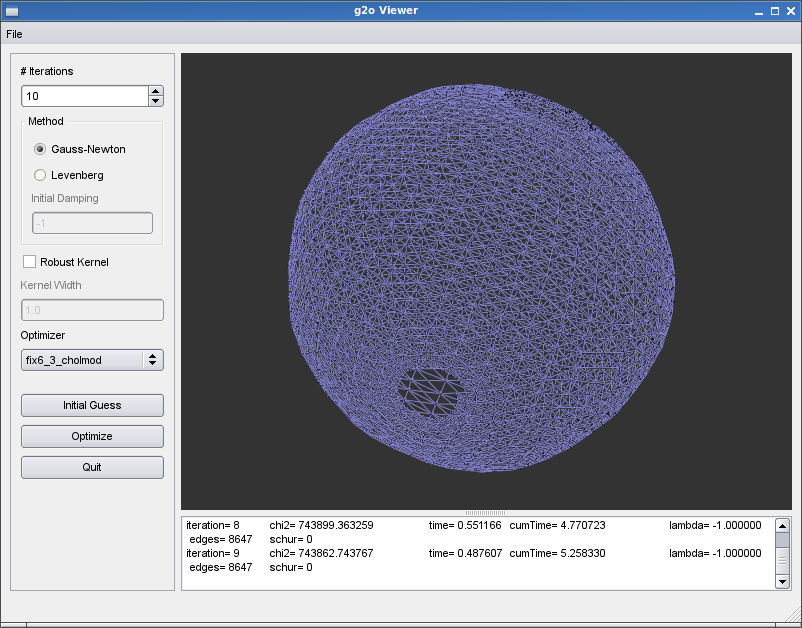g2o的图形界面。 GUI允许选择不同的合适优化器并执行优化。

g2o增量(incremental)
g2o包括用于以增量方式执行优化的实验二进制，即，在插入一个或多个节点及其测量之后进行优化。 在这种情况下，g2o在Hessian矩阵上执行ranke更新以更新线性方程组。 有关其他信息，请参阅g2o_incremental子文件夹中的README。

g2o_incremental的文件夹应该指的是当前g2o目录下的，g2o/examples/interactive_slam/g2o_incremental文件夹

该README文件的内容如下：
g2o_incremental implements a variant of the iSAM algorithm.
In contrast to iSAM the update of the Cholesky factor is done
using rank updates as provided via the CHOLMOD library.

“iSAM: Incremental Smoothing and Mapping”
by M. Kaess, A. Ranganathan, and F. Dellaert.
IEEE Trans. on Robotics, vol. 24, no. 6, Dec. 2008, pp. 1365-1378

Furthermore, it implements a simple protocol to control the operation of the
algorithm. See protocol.txt for more details or
http://slameval.willowgarage.com/workshop/submissions/ for an evaluation system
as well as data sets.

In the standard configuration g2o_incremental solves after inserting 10 nodes.
This behavior can be modified via a command line option. Furthermore, by
specifying -g on the command line a gnuplot instance is created to visualize
the graph.

Please note that both the visualization via Gnuplot and the verbose output
affect the timing results.

g2o_incremental is licensed under GPLv3.

快速看一遍后大概知道的是g2o_incremental是实现了iSAM的变体，它通过CHOLMOD库使用rank updates而不是Cholesky factor，而且实现了简单控制算法操作的协议。好了这部分看上面的文件内容，大致能了解许多。
g2o_incremental使用示例
Manhattan3500数据集的示例：


Plug-in Architecture(插件架构)
这两个工具都支持在动态库中从运行时加载类型和优化算法。

这两个工具老实说我还没搞明白作者表达的意思，我觉得可能是指g2o Viewer和g2o命令行

这实现如下。 这些工具从libs文件夹加载所有匹配* types *和* solver *的库，分别用于注册类型和优化算法。 我们假设通过加载库，类型和算法通过它们各自的构造函数注册到方程组。 清单1显示了如何向系统注册类型，清单2是一个示例，它显示了如何通过插件架构注册优化算法。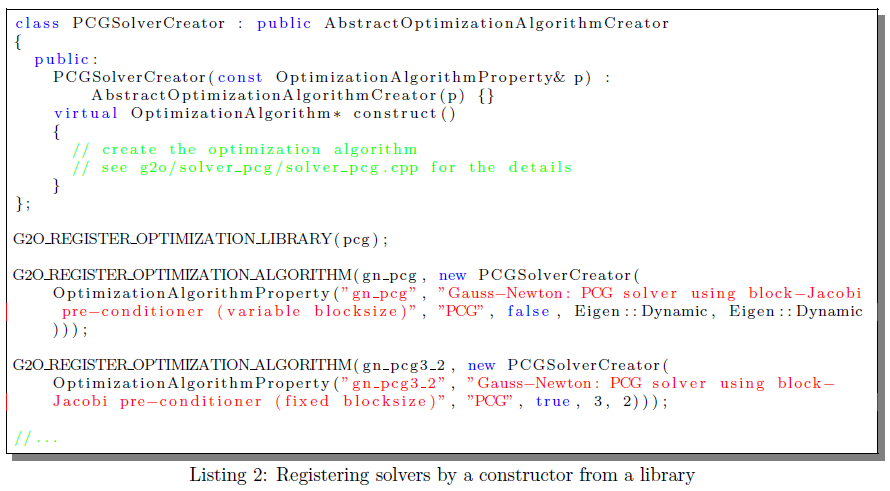对于加载包含类型或优化算法的动态库，我们支持两种不同的方法：

这些工具识别命令行开关-typeslib和-solverlib以加载特定库。
您可以指定在开始时扫描的环境变量G2O_TYPES_DIR和G2O_SOLVER_DIR，并自动加载与* types *和* solver *匹配的库。

2D SLAM:一个例子
SLAM是机器人技术中众所周知的问题，这个缩写代表“同步定位与建图”。问题可以表述如下：给定一个配备有传感器的移动机器人，我们想要从传感器测量值估计环境中机器人的地图和姿势。
通常，传感器可以分为外部感受型或本体感受型。外部感知传感器是一种测量相对于机器人移动环境的数量的装置。这些传感器的例子可以是在特定位置获取世界图像的相机，测量机器人周围的一组距离的激光扫描仪或存在重力的加速度计，其测量重力矢量或通过观察已知卫星的星座得出位姿估计的GPS。
相比之下，本体感受器传感器测量相对于先前机器人位置的机器人状态（位置）的变化。示例包括里程表，其测量机器人在两个时间步长或陀螺仪之间的相对运动。在传统的SLAM方法中，与EKF一样，这两个传感器在系统中扮演着截然不同的角色。本体感受测量用于演化一组状态变量，而外感测量用于通过反馈测量误差来校正这些估计。这不是平滑方法（如可以用g2o实现的方法）的情况，其中所有测量都以基本相似的方式处理。
SLAM的完整解决方案通常相当复杂，涉及处理原始传感器数据并确定先前看到的环境部分与实际测量（数据关联）之间的对应关系。 描述问题的完整解决方案超出了本文的范围。 但是，请注意，我们将提供一个简化但有意义的问题版本，其中包含所有相关元素，非常适合用g2o实现。
场景(scenario)是机器人在平面上移动。 机器人配备有测距传感器，能够测量机器人在两个时间帧之间的相对运动，以及“地标”传感器，其能够测量机器人参考系中机器人附近的一些环境标志的位置。 例如，可以通过从激光扫描中提取角点或通过从立体图像对中检测相关特征的位置来实现该地标检测器。 我们在本节中进行的简化是地标是唯一可识别的。 换句话说，每当机器人看到一个地标时，它就可以判断它是否是一个新的，或者它已经知道在何时是否已经看到它。
显然，里程计和地标传感器都受到噪音的影响。 原则上，如果测距不会受到噪声的影响，则可以简单地通过连接测距测量来重建机器人的轨迹。 然而，情况并非如此，并且集成测距法导致增加的定位误差，当机器人重新进入已知区域时该定位误差变得明显【回环检测能约束该误差】。 以类似的方式，如果机器人具有无限的感知范围，则它可以一次获取所有地图并且可以通过简单的几何构造来检索位置。 同样情况并非如此，并且机器人感知位于最大范围内的地标的位置。 这些测量受到噪声的影响，噪声通常随着机器人的地标距离而增加。
下面将介绍在g2o中表征问题所需的所有必要步骤。 这些是：

识别状态变量${\rm x}_i$及其定义域，
约束和图结构的识别的表征，
增量$\Delta\tilde{\rm x}_i$的参数化，以及$\boxplus$操作符的定义。
误差函数${\rm e}_k({\rm x}_k)$的构造

确定状态变量
下图示出了SLAM图的片段。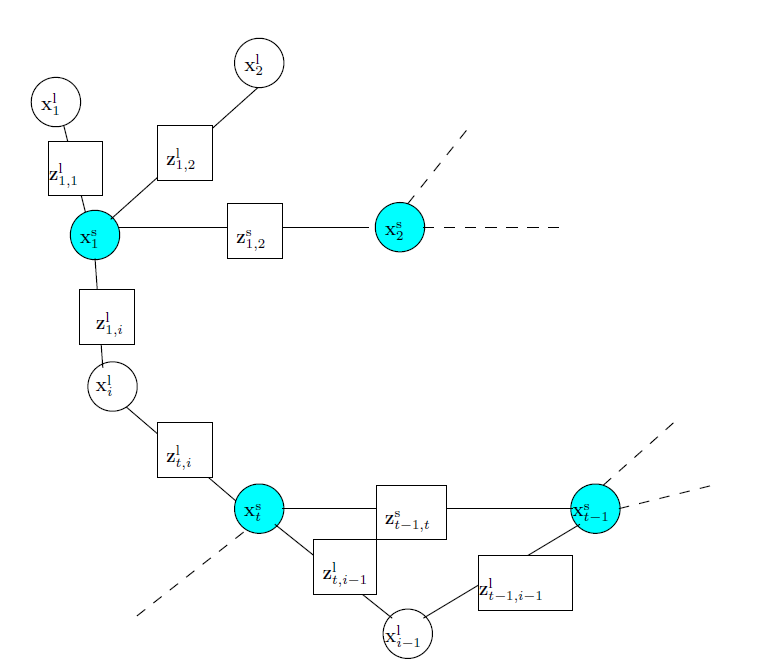SLAM过程的图形表示。 用圆形节点描绘的图的顶点表示机器人位姿${\rm x}_*^{\rm s}$或地标${\rm x}_*^{\rm l}$。 通过约束${\rm z}_*^{\rm l}$捕获来自机器人位姿的地标的测量，并且通过约束${\rm z}_*^{\rm s}$对连接后续机器人姿势的测距测量进行建模。

机器人位置由节点${\rm x}_t^{\rm s}$表示，而地标由节点${\rm x}_i^{\rm l}$表示。 我们假设我们的地标传感器只能检测地标的2D位姿，而不能检测其方向。 换句话说，地标"活"在$\mathfrak{R}^2$，机器人位姿由平面上的机器人位置$（x，y）$及其方向$\theta$参数化，因此它们属于2D变换群$SE(2)$。 更正式地说，2D SLAM图的节点有两种类型

机器人位置：${\rm x}_t^{\rm s}=\begin{pmatrix}x_t^{\rm s}&y_t^{\rm s}&\theta_t^{\rm s}\end{pmatrix}^T\in SE(2)$
地标位置：${\rm x}_i^{\rm l}=\begin{pmatrix}x_i^{\rm l}&y_i^{\rm l}\end{pmatrix}^T\in \mathfrak{R}^2$

约束的建模
两个随后的机器人位姿${\rm x}_t^{\rm s}$和${\rm x}_{t+1}^{\rm s}$通过测距法测量相关联，其表示通过测距法测量的机器人从${\rm x}_t^{\rm s}$到${\rm x}_{t+1}^{\rm s}$的相对运动。 由于影响传感器的噪声，该测量通常与两个位姿之间的实际变换略有不同。 作为里程测量，欧氏变换，它也是$SE(2)$群的成员。 假设影响测量的噪声是白噪声和高斯噪声，它可以通过$3×3$对称正定信息矩阵建模。 在实际应用中，该矩阵的元素取决于机器人的运动。 即，运动越大，不确定性就越大。 因此，节点${\rm x}_t^{\rm s}$和${\rm x}_{t+1}^{\rm s}$之间的测距边缘由这两个实体组成：

${\rm z}_{t,t+1}^{\rm s}\in SE(2)$表示节点之间的运动以及
$\Omega_{t,t+1}^{\rm s}\in \mathfrak{R}^{3\times 3}$表示测量的逆协方差(the inverse covariance of the measurement)，因此是对称的和正定的。

如果机器人从位置${\rm x}_i^{\rm l}$感测到地标${\rm x}_t^{\rm s}$，则相应的测量将建模为由从机器人位姿到路标的边。 关于地标的测量包括在机器人系中感知的x-y平面中的点。 因此，具有里程碑意义的测量值就像地标一样存在于$\mathfrak{R}^2$中。 同样，在白高斯噪声假设下，噪声可以通过其逆协方差来建模。 因此，机器人位姿和地标之间的边以这种方式被参数化：

${\rm z}_{t,i}^{\rm l}\in \mathfrak{R}^2$表示由${\rm x}_t^{\rm s}$表示的坐标系中的地标的位置和
$\Omega_{t,i}^{\rm l}\in \mathfrak{R}^{2\times 2}$表示测量的逆协方差，是SPD。

SPD:Symmetric positive definite,对称且正定。作者这个缩写真的是秀了。

增量参数化的选择
到目前为止，我们已经定义了使用g2o实现2D SLAM算法所需的大部分元素。 即，我们描述了变量的定义域和测量的定义域。 剩下要做的是为我们系统中的两种边定义误差函数，并确定增量的（可能是智能的）参数化。
地标位置参数化为$\mathfrak{R}^2$，它已经是欧几里德空间。 因此，增量$\Delta\tilde{\rm x}_i^{\rm l}$可以存在于同一空间中，并且可以安全地选择$\boxplus$运算符作为向量和：
${\rm x}^\mathrm{l}_i \boxplus \Delta\tilde{\rm x}^\mathrm{l}_i \doteq {\rm x}^\mathrm{l}_i + \Delta\tilde{\rm x}^\mathrm{l}_i$
相反，这些位姿活在(live in,你可以认为是属于)非欧几里德空间$SE(2)$中。 此空间允许许多参数化。 示例包括：旋转矩阵${\mathbf R}(\theta)$和平移向量$(x\;y)^T$或角度$\theta$和平移向量$(x\;y)^T$。
作为增量的参数化，我们选择最小的一个，即平移向量和角度。 选择此参数化后，我们需要在位姿和位姿增量之间定义$\boxplus$运算符。 一种可能的选择是将三个标量参数$x$，$y$和位姿的$\theta$视为矢量，并将$\boxplus$定义为矢量和。 这是一个糟糕的选择有很多原因。 其中之一是角度不是欧几里德，人们需要在每次添加后重新归一化(re-normalize)它们。
更好的选择是将位姿和位姿增量之间的$\boxplus$定义为运动合成运算符。 即，给定机器人位姿${\rm x}_t^{\rm s}=\begin{pmatrix}x&y&\theta\end{pmatrix}^T$和增量$\Delta\tilde{\rm x}_t^{\rm s}=\begin{pmatrix}\Delta x&\Delta y&\Delta\theta\end{pmatrix}^T$，其中$\Delta x$是纵向位移（即，在机器人的航向方向上），$\Delta y$是横向位移和$\Delta\theta$是旋转变化 ，运算符可以定义如下：
${\rm x}^\mathrm{s}_t \boxplus \Delta\tilde{\rm x}^\mathrm{s}_t \doteq ​ \left( ​ \begin{array}{c} ​ x + \Delta x \cos \theta - \Delta y \sin \theta \\ ​ y + \Delta x \sin \theta + \Delta y \cos \theta \\ ​ \mathrm{normAngle}(\theta + \Delta \theta) ​ \end{array} ​ \right)\tag{36}$
​    $= {\rm x}^\mathrm{s}_t \oplus \Delta\tilde{\rm x}^\mathrm{s}_t\tag{37}$
在前面的等式中，我们引入了运动合成运算符$\oplus$与$\oplus$类似，有$\ominus$运算符执行相反的操作，定义如下：
${\rm x}^\mathrm{s}_a \ominus {\rm x}^\mathrm{s}_b \doteq ​ \left( ​ \begin{array}{c} ​ (x_a - x_b) \cos \theta_b + (y_a - y_b) \sin \theta_b \\(x_a - x_b) \sin \theta_b + (y_a - y_b) \cos \theta_b \\ \mathrm{normAngle}(\theta_b - \theta_a) ​ \end{array} ​ \right)\tag{38}$
误差函数的设计
形式化问题的最后一步是设计“合理”的误差函数${\rm e}({\rm x}_k)$。 一种常见的方法是定义一个所谓的测量函数${\mathbf h}_k({\rm x}_k)$，给定集合${\rm x}_k$中顶点的先验，它能“预测”测量$\hat{\rm z}_k$。 定义此函数通常相当简单，可以通过直接实现误差模型来完成。 随后，可以将误差矢量计算为预测$\hat{\rm z}_k$和实际测量之间的矢量差。 这是构造误差函数的一般方法，当误差的空间位于测量原点周围的局部欧几里得时，它就起作用。 如果不是这种情况，可能想要用更“常规”的其他运算符替换向量差。
我们现在将构建连接机器人位姿${\rm x}_t^{\rm s}$和地标${\rm x}_i^{\rm l}$的边的误差函数。 第一步是构建计算“虚拟测量”的测量预测${\mathbf h}_{t,i}^{\mathrm l}({\rm x}_t^{\rm s}，{\rm x}_i^{\rm l})$。 该虚拟测量是从机器人位姿${\rm x}_t^{\rm s}$看到的地标${\rm x}_i^{\rm l}$的位置。 ${\mathbf h}_{t,i}^{\mathrm l}(\cdot)$的等式如下：
${\mathbf h}^\mathrm{l}_{t,i}({\rm x}^\mathrm{s}_t, {\rm x}^\mathrm{l}_i) \doteq \left(\begin{array}{c} (x^\mathrm{s}_t - x_i) \cos\theta^\mathrm{s}_t + (y^\mathrm{s}_t - y_i) \sin \theta^\mathrm{s}_t \\-(x^\mathrm{s}_t - x_i) \sin \theta^\mathrm{s}_t + (y^\mathrm{s}_t - y_i) \cos\theta^\mathrm{s}_t \end{array}\right)\tag{39}$
它将地标的位置转换为机器人的坐标系。
由于地标位于欧几里德空间中，因此将误差函数计算为法向量差是合理的。 这导致以下关于地标的误差函数的定义：
$​ {\rm e}^\mathrm{l}_{t,i}({\rm x}^\mathrm{s}_t, {\rm x}^\mathrm{l}_i) \doteq {\rm z}_{t,i} - {\rm h}^\mathrm{l}_{t,i}({\rm x}^\mathrm{s}_t, {\rm x}^\mathrm{l}_i)\tag{40}$
以类似的方式，我们可以定义连接两个机器人位姿${\rm x}_t^{\rm s}$和${\rm x}_{t+1}^{\rm s}$的测距边的误差函数。 如前所述，测距法测量存在于$SE(2)$中。 通过使用$\oplus$运算符，我们可以编写一个综合测量函数：
$​ {\rm h}^\mathrm{s}_{t,t+1}({\rm x}^\mathrm{s}_t, {\rm x}^\mathrm{s}_{t+1}) \doteq {\rm x}^\mathrm{s}_{t+1} \ominus {\rm x}^\mathrm{s}_{t}\tag{41}$
简而言之，此函数返回机器人从${\rm x}_t^{\rm s}$到${\rm x}_{t+1}^{\rm s}$的运动，即“理想”的测距。 而且可以再次获得误差，误差作为测量和预测之间的差异。 但是，由于我们的测量不存在于欧几里德空间，我们可以使用$\ominus$而不是矢量差:
${\rm e}^\mathrm{s}_{t,t+1}( {\rm x}^\mathrm{s}_t, {\rm x}^\mathrm{s}_{t+1}) \doteq {\rm z}_{t,t+1} \ominus {\rm h}^\mathrm{s}_{t,t+1}({\rm x}^\mathrm{s}_t, {\rm x}^\mathrm{s}_{t+1})\tag{42}$
整理(Putting things together)
在这里，我们总结了先前问题定义的相关部分，并为实现做好了准备。

变量
符号
定义域
维数
$\Delta {\rm x}$的参数化
$\boxplus$运算符

相机位姿
${\rm x}_t^{\rm s}$
$SE(2)$
$3$
$\begin{pmatrix}\Delta{\rm x}&\Delta{\rm y}&\Delta\theta\end{pmatrix}$
${\rm x}_t^{\rm s}\oplus\Delta{\rm x}_t^{\rm s}$

地标位姿
${\rm x}_i^{\rm l}$
$\mathfrak{R}(2)$
2
$\begin{pmatrix}\Delta{\rm x}&\Delta{\rm y}\end{pmatrix}$
${\rm x}_i^{\rm l}+\Delta{\rm x}_i^{\rm l}$

测量
符号
定义域
维数
涉及的变量集${\rm x}_k$
误差函数

里程计
$={\rm z}_{t,t+1}^{\rm s}$
$SE(2)$
3
$\{{\rm x}_t^{\rm s},{\rm x}_{t+1}^{\rm s}\}$
${\rm e}^\mathrm{s}_{t,t+1}( {\rm x}^\mathrm{s}_t, {\rm x}^\mathrm{s}_{t+1}) \doteq {\rm z}_{t,t+1} \ominus {\rm h}^\mathrm{s}_{t,t+1}({\rm x}^\mathrm{s}_t, {\rm x}^\mathrm{s}_{t+1})$

路标
$={\rm z}_{t,i}^{\rm l}$
$\mathfrak{R}(2)$
2
$\{{\rm x}_t^{\rm s},{\rm x}_i^{\rm l}\}$
${\rm e}^\mathrm{l}_{t,i}({\rm x}^\mathrm{s}_t, {\rm x}^\mathrm{l}_i)\doteq {\rm z}_{t,i} - {\rm h}^\mathrm{l}_{t,i}({\rm x}^\mathrm{s}_t, {\rm x}^\mathrm{l}_i)$

我们要做的第一件事是实现一个表示$SE(2)$群元素的类。 我们通过使用旋转矩阵和平移向量表示，通过Eigen :: Geometry中定义的类型在内部表示这些元素。 因此，我们定义了一个实现运动合成运算符$\oplus$的operator *（...），以及一个返回转换逆的inverse()函数。 为方便起见，我们还实现了一个转换2D点的operator*。 为了将元素转换为使用Eigen :: Vector3d的最小表示，我们定义了fromVector（...）和toVector（...）方法。
构造函数将此类初始化为原点中的面向0度的一个点。

原句是：The constructor initializes this class as a point in
the origin oriented at 0 degrees.这句翻译起来和理解起来让我有点理解困难，大体是是构造函数初始化该类为一个点。然后这个点在原点，朝向0°的方向。其实意思在代码中比较明显，就是$x,y=0，\theta=0^\circ$

请注意，在g2o中，为群创建单独的类不是必需的，但使代码更具可读性和可重用性。 清单3中报告了相应的C ++类。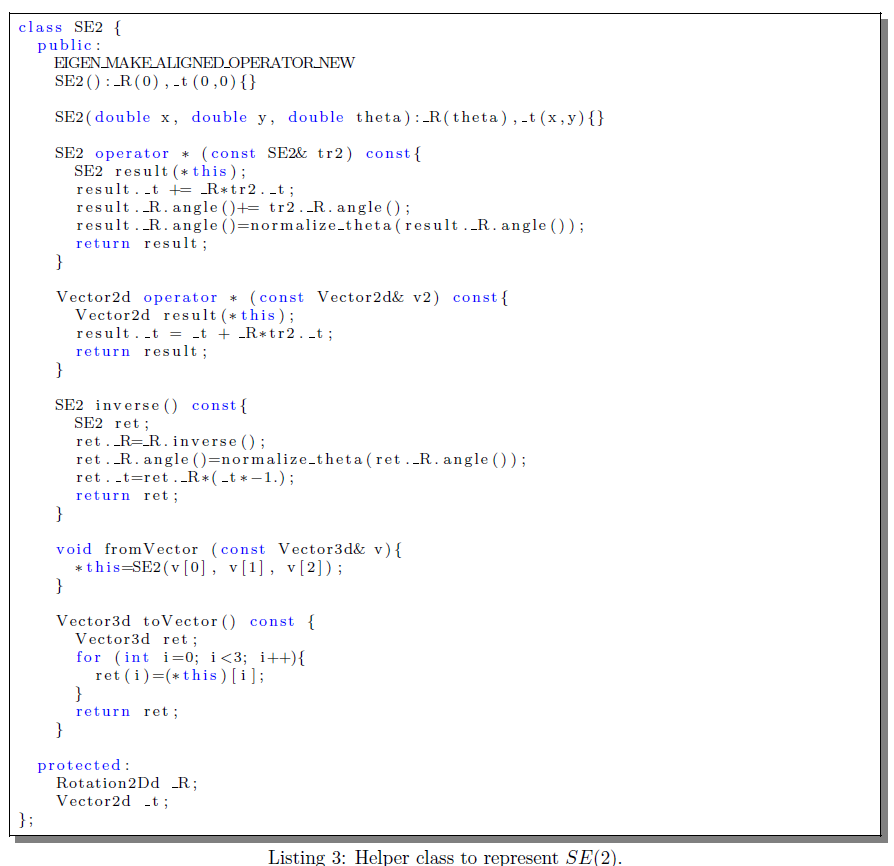一旦我们定义了很好的$SE(2)$群，我们就可以实现顶点了。为此，我们扩展了BaseVertex <>类，我们派生了VertexSE2类来表示机器人位姿，并使用VertexPointXY来表示平面中的点地标。清单4中报告了机器人姿势顶点的类定义。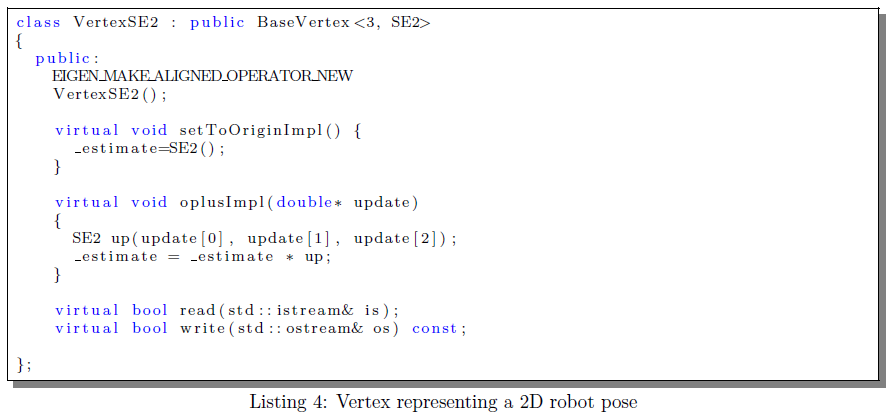位姿顶点(pose-vertex)扩展了BaseVertex <>的模板特化。我们应该对g2o说内部类型的维度为$3$，估计值为SE2。这意味着VertexSE2的成员_estimate是SE2类型。然后我们需要做的就是重新定义setToOriginImpl()方法，将估计重置为已知配置，方法为oplusImpl（double *）。该方法应该应用一个增量，用增量参数化表示（即向量$\begin{pmatrix}\Delta{\rm x}&\Delta{\rm y}&\Delta\theta\end{pmatrix}^t$到当前估计。为此，我们首先将作为参数传递的向量转换为SE2，然后我们将此增量相乘在之前的估计的右边。之后我们应该对流实现读写函数，但这很简单，你可以在代码中自己查找。

bool VertexSE2::read(std::istream& is)
{
Eigen::Vector3d p;
is >> p >> p >> p;
_estimate.fromVector(p);
return true;
}

bool VertexSE2::write(std::ostream& os) const
{
Eigen::Vector3d p = estimate().toVector();
os << p << " " << p << " " << p;
return os.good();
}


所示的例子在g2o目录下的g2o/example/tutorial_slam2d文件夹下

下一步是实现顶点来描述地标位置。 由于地标在$\mathfrak{R}(2)$中被参数化，我们不需要为此定义任何群，并且我们直接使用在Eigen中定义的向量类。 清单5中报告了此类。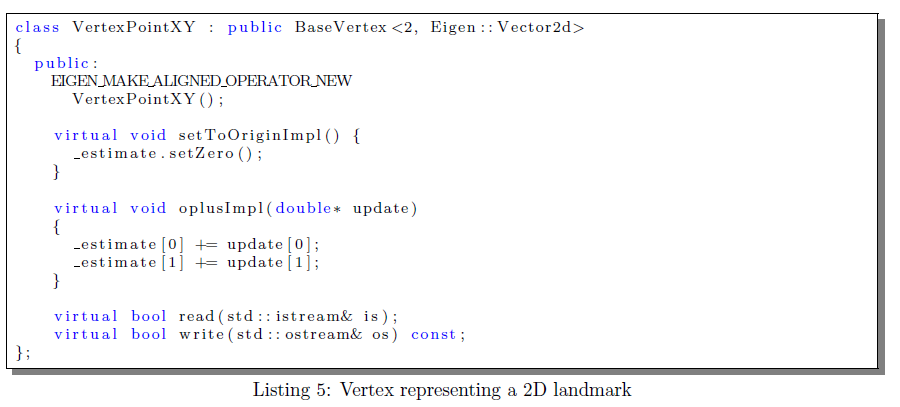现在我们完成了顶点。 我们应该来完成边。 由于两个边都是二元边，我们扩展BaseBinaryEdge<>类。 为了表示连接两个VertexSE2的测距边缘（参见清单6），我们需要扩展BaseBinaryEdge <>，专门用于连接顶点的类型（顺序很重要），其中测量本身由维度为$3$的SE2表示 。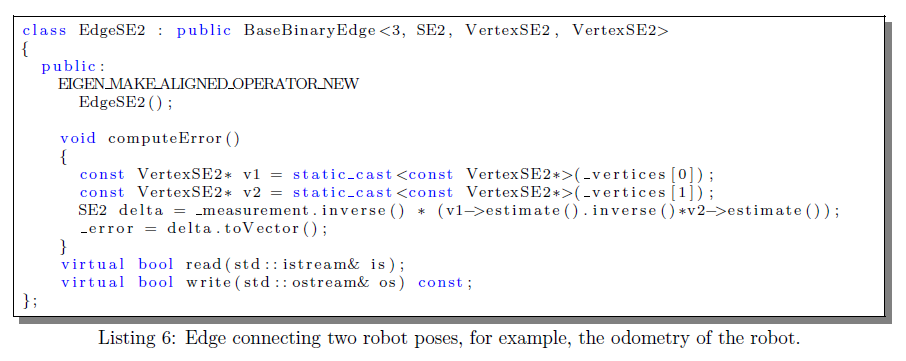第二个模板参数是用于成员变量_measurement的参数。 第二步是通过重新定义computeError()方法来构造误差函数。computeError()应该将误差向量放在具有类型Eigen :: Vector <double，3>的成员变量error中。 这里的3来自指定维度的模板参数。 同样，可以在代码中查找读写函数。
    bool EdgeSE2::read(std::istream& is)
{
Vector3d p;
is >> p >> p >> p;
_measurement.fromVector(p);
_inverseMeasurement = measurement().inverse();
for (int i = 0; i < 3; ++i)
for (int j = i; j < 3; ++j) {
is >> information()(i, j);
if (i != j)
information()(j, i) = information()(i, j);
}
return true;
}

bool EdgeSE2::write(std::ostream& os) const
{
Vector3d p = measurement().toVector();
os << p.x() << " " << p.y() << " " << p.z();
for (int i = 0; i < 3; ++i)
for (int j = i; j < 3; ++j)
os << " " << information()(i, j);
return os.good();
}

现在我们完成了边的构造。Jacobians用g2o数值计算（默认实现）。 但是，如果您希望在一切正常后加快代码执行速度，则会可以重新定义linearizeOplus方法。
剩下要做的最后一件事是定义一个类来表示一个地标的测量。 在清单7中被显示。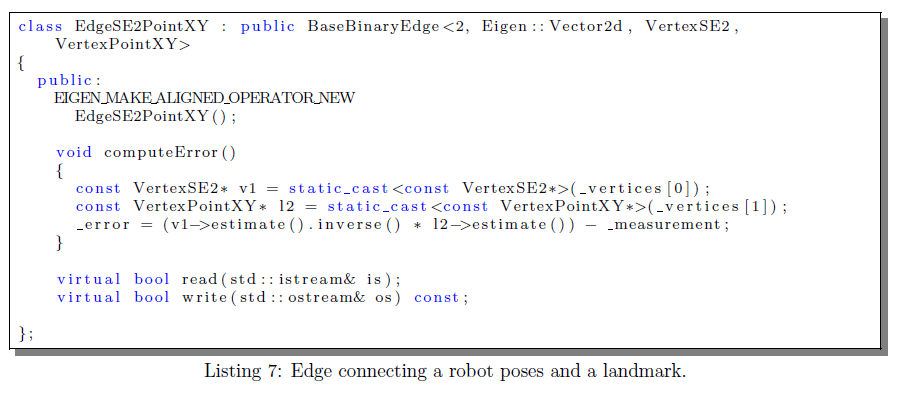我们再次扩展了BaseBinaryEdge <>的特化，我们告诉系统它将VertexSE2与VertexPointXY连接，测量由具有维度大小为$2$的Eigen :: Vector2d表示。
我们应该做的最后一步是让我们的系统运行，就是注册类型让g2o知道有新类型准备就绪。 但是，如果您打算手动构建图而不在磁盘上执行任何$\rm i/o$操作，则甚至不需要执行此步骤。
总结
通过阅读g2o的官方文献，基本大体知道了应该这么使用，下一节运行和调试example,如果有时间，可能看看g2o适不适合写教程。不过目前来说，我时间不算很多。只能尽力看情况，能不能把自己学习过程记录下来了。

: g2o: A general Framework for (Hyper) Graph Optimization，March 11, 2017
:  SLAM 十四讲，第六章
: Probabilistic Robotics，chapter 11，2005
​


展开全文最小二乘 2D SLAM
• g2o学习记录（2）官方文档的阅读及理解 深入理解图优化与g2og2o篇 https://blog.csdn.net/u014365862/article/details/52716538 graph slam学习:g2o https://blog.csdn.ne...
https://blog.csdn.net/YuYunTan/article/details/85255345

g2o学习记录（2）官方文档的阅读及理解

深入理解图优化与g2o：g2o篇

https://blog.csdn.net/u014365862/article/details/52716538

graph slam学习:g2o

https://blog.csdn.net/xyz599/article/details/53436544

g2o小记

https://blog.csdn.net/u010566411/article/details/72904266  仿真，矩形轨迹

深入理解图优化与g2o：g2o篇

http://www.cnblogs.com/gaoxiang12/p/5244828.html

一起做RGB-D SLAM (6)

第六讲 图优化工具g2o的入门

https://www.cnblogs.com/gaoxiang12/p/4739934.html


展开全文• 最近 因为工作原因 终于 比较细致地阅读了下 g2o源码， 先写了个word文档 ，应该是 网上比较详细的了 后面上传到 下载资源中
最近 因为工作原因 终于 比较细致地阅读了下 g2o源码， 先写了个word文档 ，应该是 网上比较详细的了
后面上传到 下载资源中
https://download.csdn.net/download/haithink/11958938?spm=1001.2014.3001.5501
从这下载


展开全文• 深入理解图优化与g2o：图优化篇 ...　关于g2o，我13年写过一个文档，然而随着自己理解的加深，越发感觉不满意。本着对读者更负责任的精神，本文给大家重新讲一遍图优化和g2o。除了这篇文档，读者还可以找到一篇关于
• 今天第三边跟着slam十四讲敲了g2o的曲线拟合代码，对g2o的认识也更进一步 ...这是ros上关于g2o库的文档，和g2o差别不大：http://docs.ros.org/fuerte/api/re_vision/html/namespaceg2o.html 进...
• g2o是视觉SLAM中常用的图优化库，该文档主要介绍其使用方法和细节。
• 文章目录图优化基本概念g2o在前端小BA 在(3D-2D)求解pnp位姿优化上的应用g2o在前端小BA 在(3D-3D)求解pnp位姿优化上的应用参考文档 图优化基本概念 g2o在前端小BA 在(3D-2D)求解pnp位姿优化上的应用 #include <...图优化
• graph slam tutorial ：从推导到应用3（g2o版程序），包含文档读取，及后端优化
• 转载自 半闲居士 在这里，感谢博主的无私分享。...　关于g2o，我13年写过一个文档，然而随着自己理解的加深，越发感觉不满意。本着对读者更负责任的精神，本文给大家重新讲一遍图优化和g2o。除了这篇文档，读优化 图优化
• ## vs2013+g2o配置

千次阅读 2017-08-03 19:03:39
注： 编译工具选择vs2013(vc12)，vs2010对于某些编译会出问题，vs2017目前...参考文档： 【文档一】http://m.blog.csdn.net/xiamentingtao/article/details/50100549（suitesparse-metics安装） 【文档二】http://blog.
• ## g2o中 EdgeSE3Expmap类型Jacobian的计算

万次阅读 热门讨论 2017-08-29 16:15:02
重新翻看Ethan Eade的《Lie Groups for 2D and 3D Transformations》，发现他的文档早已有相关推导。比如针对两个SO3乘积对其中一个求导： 比如同理两个SE3乘积对其中一个求导： 上面这两个jacobian
• 提示：文章写完后，目录可以自动生成，如何生成可参考右边的帮助文档 文章目录前言一、pandas是什么？二、使用步骤1.引入库2.读入数据总结 前言 提示：这里可以添加本文要记录的大概内容： 例如：随着人工智能的...
• 文档生成工具 APIDOC 笔记简介代码即文档，代码注释生成文档是一种很好的文档方式。通过文档的时序化更新可以追溯整个工程的历史进程。...入门1、安装命令npm install apidoc -g2、运行命令apidoc -i myapp/ -o apidoc/注释 语言
• 文档(用文档表示是否合适??)详细介绍中国电信的短信网关（SMG应为SMGW,为统一起见,建议所有的短信网关改为短消息网关,所有的短信中心改为短消息中心）和服务提供商（SP）之间、短信网关和短信网关之间的通信协议。...
• 非线性曲线拟合，高博士给的demo主要用谷歌ceres库实现，高斯牛顿方式实现，g2o库实现，这三个程序例子。 一.首先介绍ceres库安装与实现 ceres库是谷歌开发的C++库，用于建模和解决复杂的优化问题的。能用于解决非...ceres
• Ceres官网上的文档非常详细地介绍了其具体使用方法，相比于另外一个在slam中被广泛使用的图优化库G2O，ceres的文档可谓相当丰富详细（没有对比就没有伤害，主要是G2O资料太少了，对比起来就显得ceres的很多），下面...
• Ceres solver 是谷歌开发的一...Ceres官网上的文档非常详细地介绍了其具体使用方法，相比于另外一个在slam中被广泛使用的图优化库G2O，ceres的文档可谓相当丰富详细（没有对比就没有伤害，主要是G2O资料太少了，对...
• Ceres官网上的文档非常详细地介绍了其具体使用方法，相比于另外一个在slam中被广泛使用的图优化库G2O，ceres的文档可谓相当丰富详细（没有对比就没有伤害，主要是G2O资料太少了，对比起来就显得ceres的很多），下面...